# West Bengal Madhyamik Board Question Paper for Class 10th Maths 2017 In PDF

West Bengal Madhyamik (Class 10) Maths 2017 question paper with solutions are available on this page by BYJU’S in downloadable pdf format and also in the text so that the students can make use of the given question papers and compare their answers for all the questions that they have solved. Maths Question Paper 2017 Class 10 is added here, which consists of all the important concepts that have to be covered for their exams. Students can solve and practise West Bengal Madhyamik (Class 10) Maths 2017 question paper to get acquainted with the expected question types for the board exams.

Students are able to access all the West Bengal board previous year Maths question papers. Students can assess their preparation level for the upcoming board exams and also score well by practising these papers.

### WBBSE Class 10th Maths Question Paper With Solutions 2017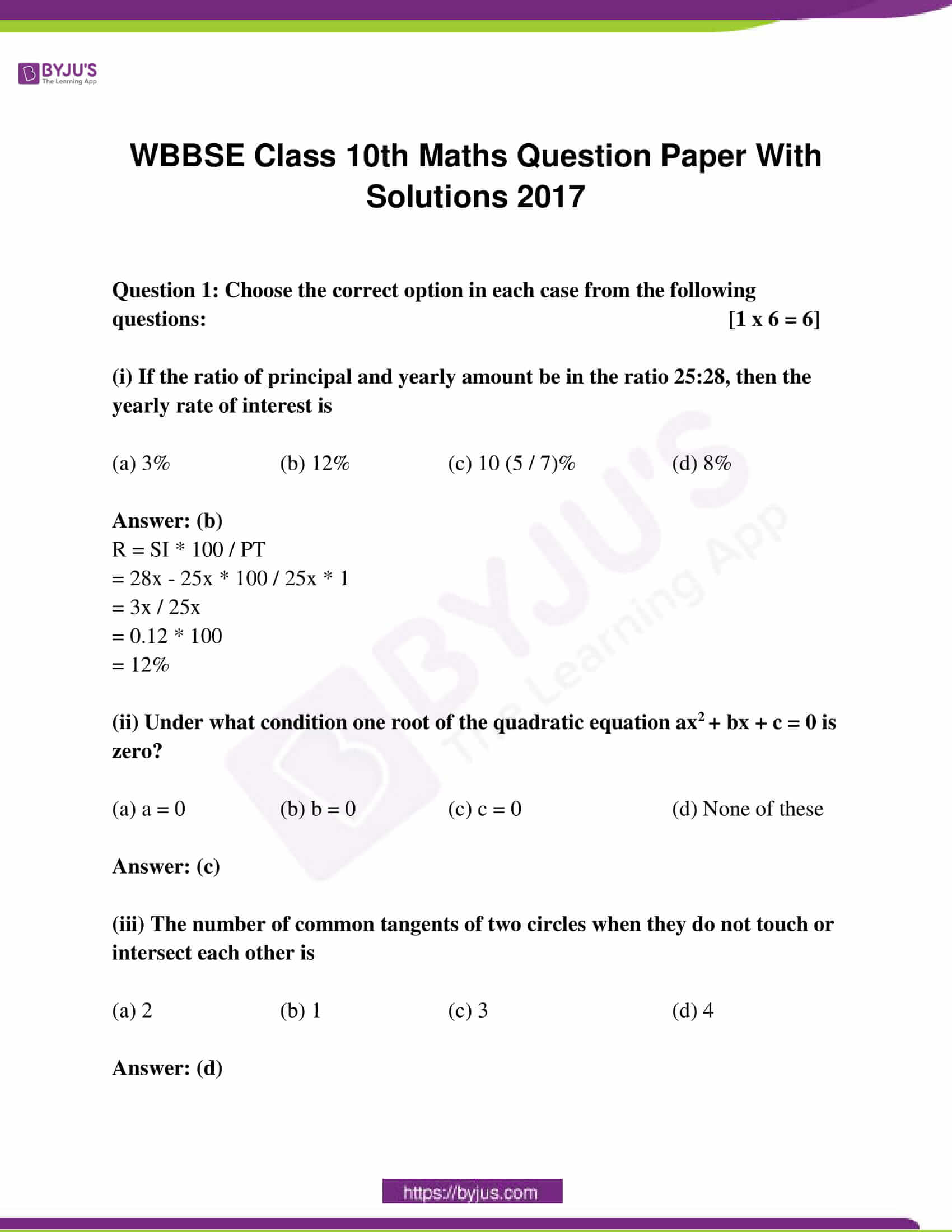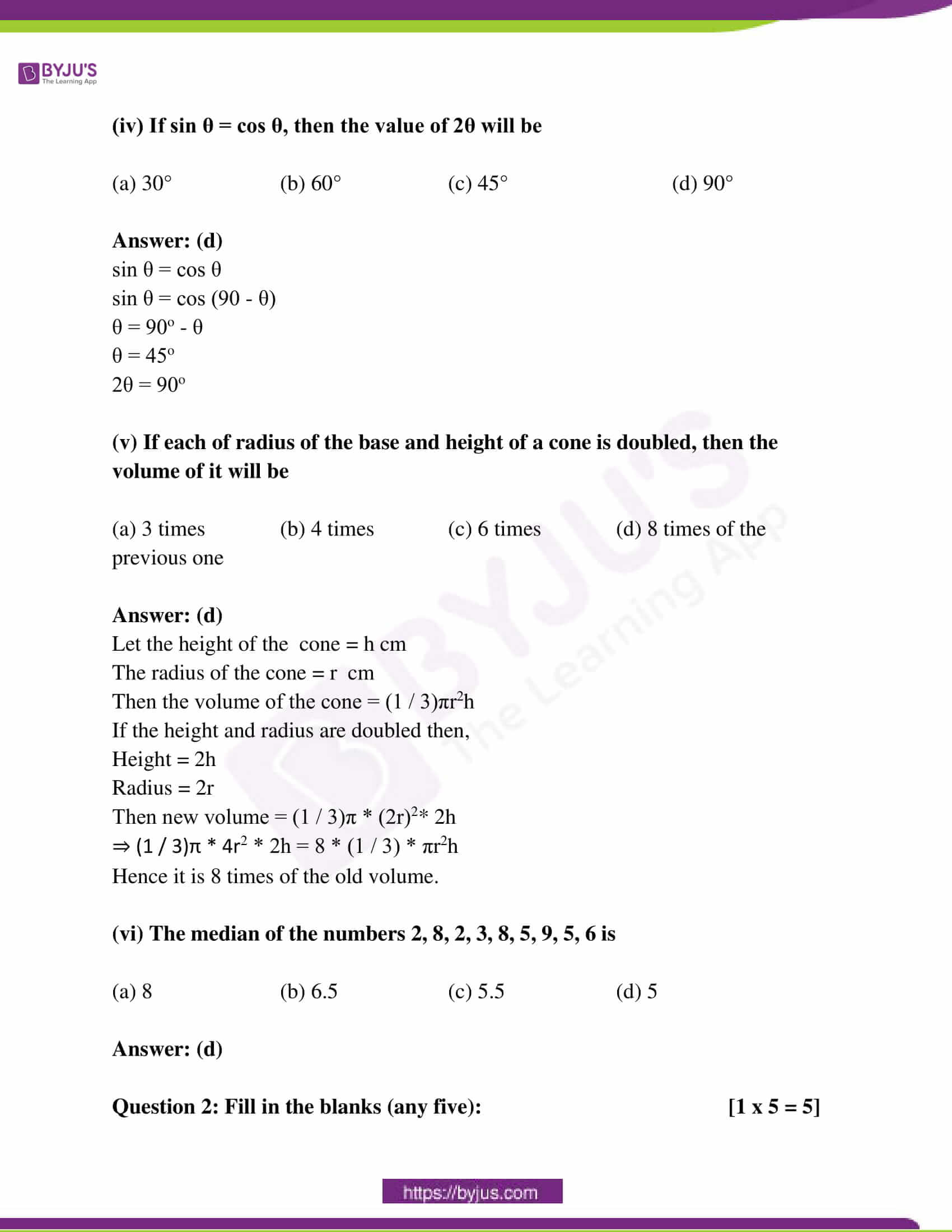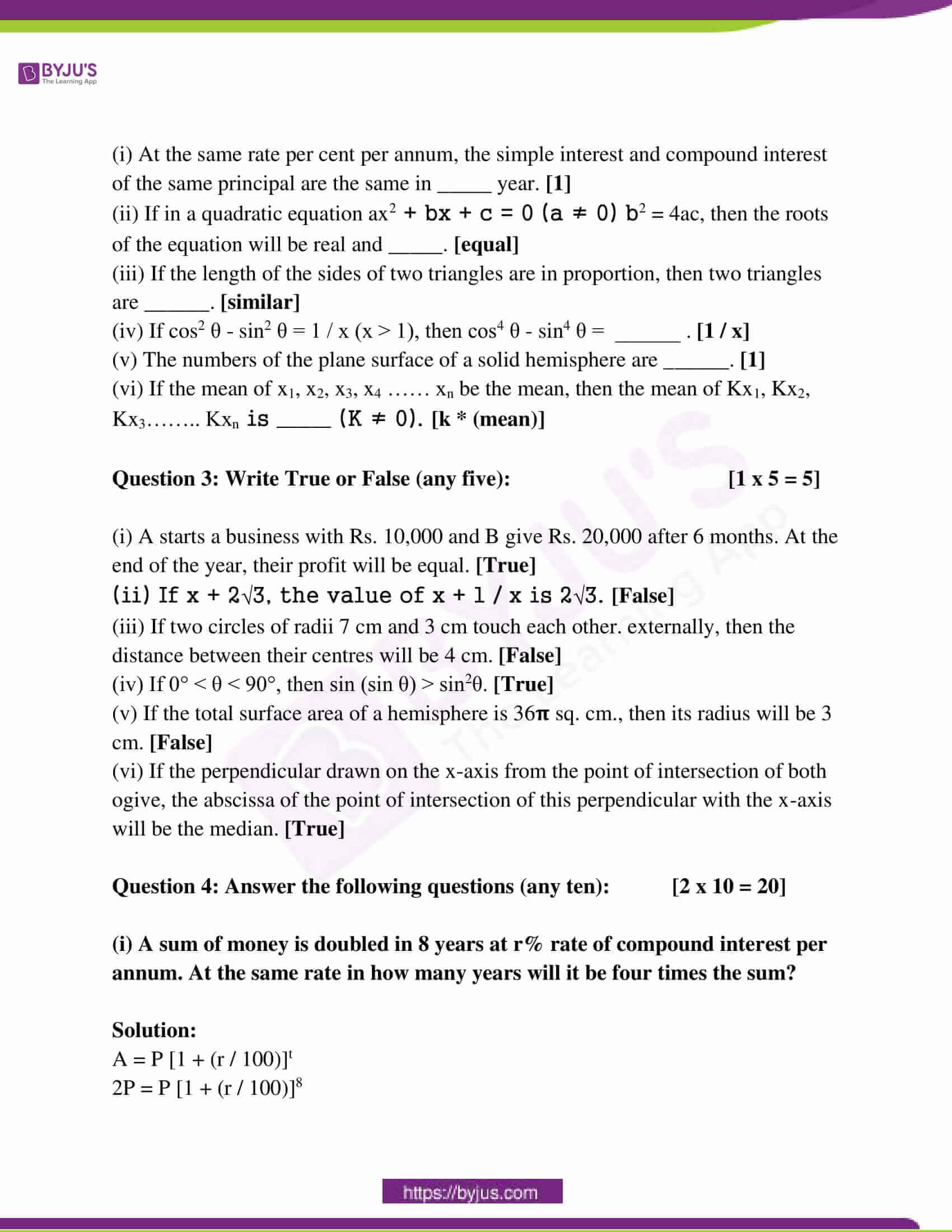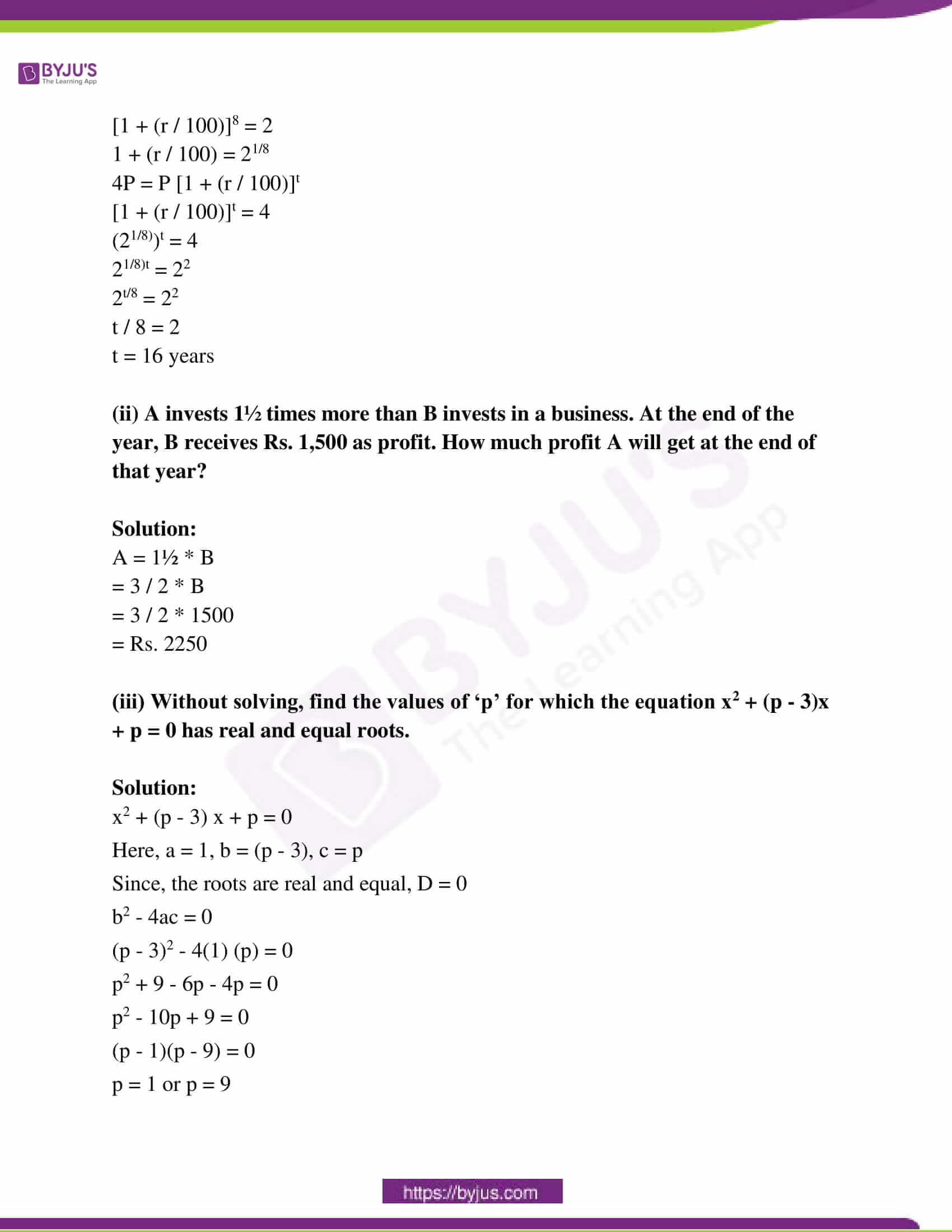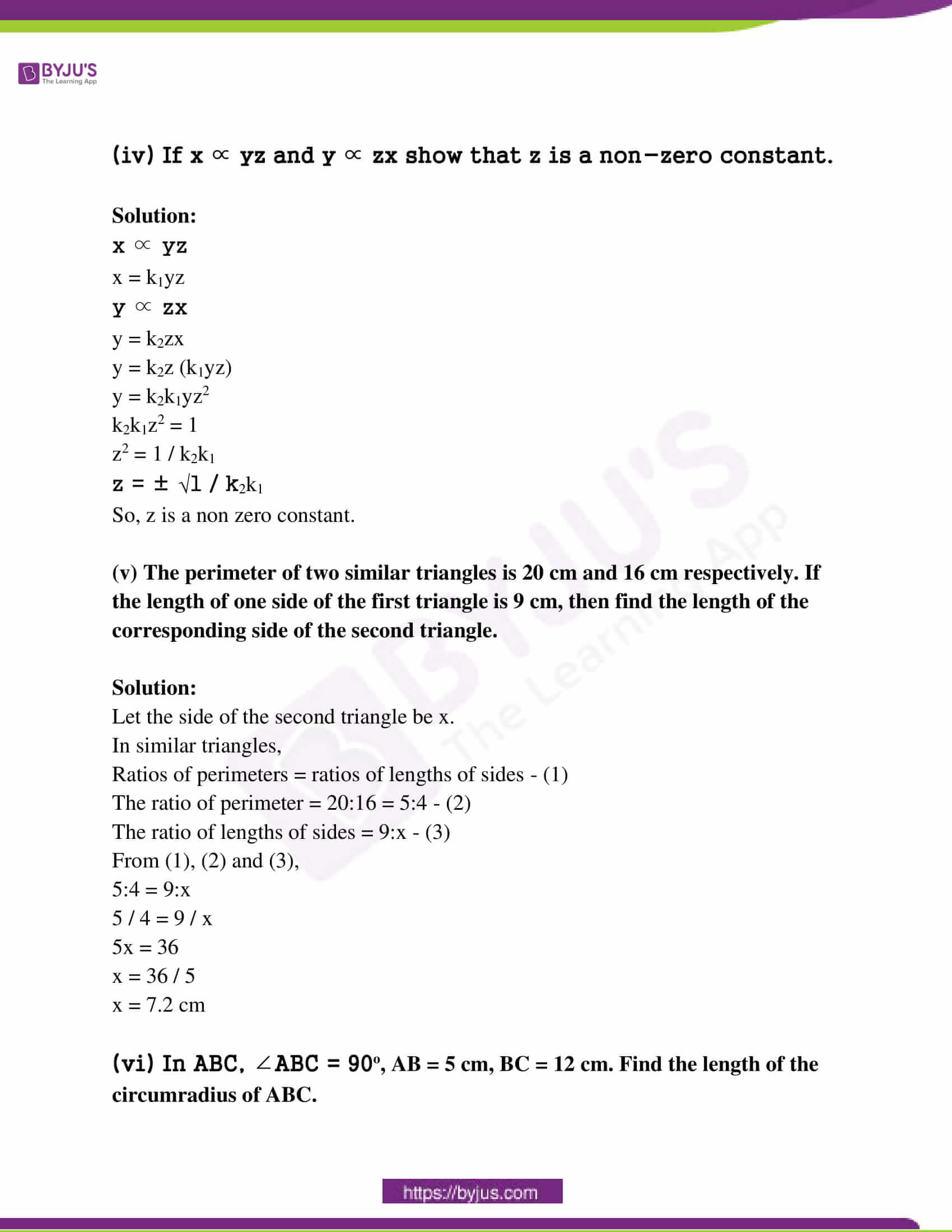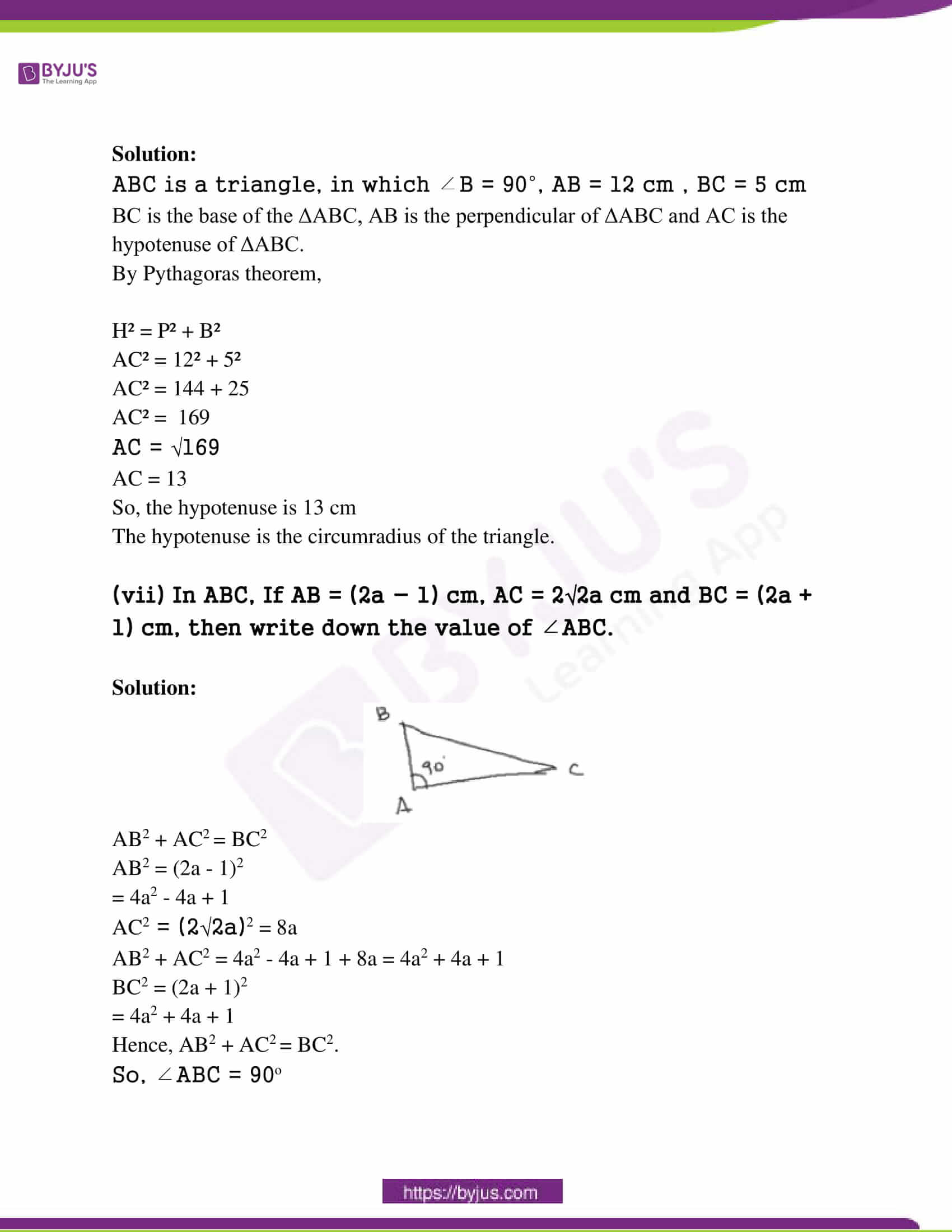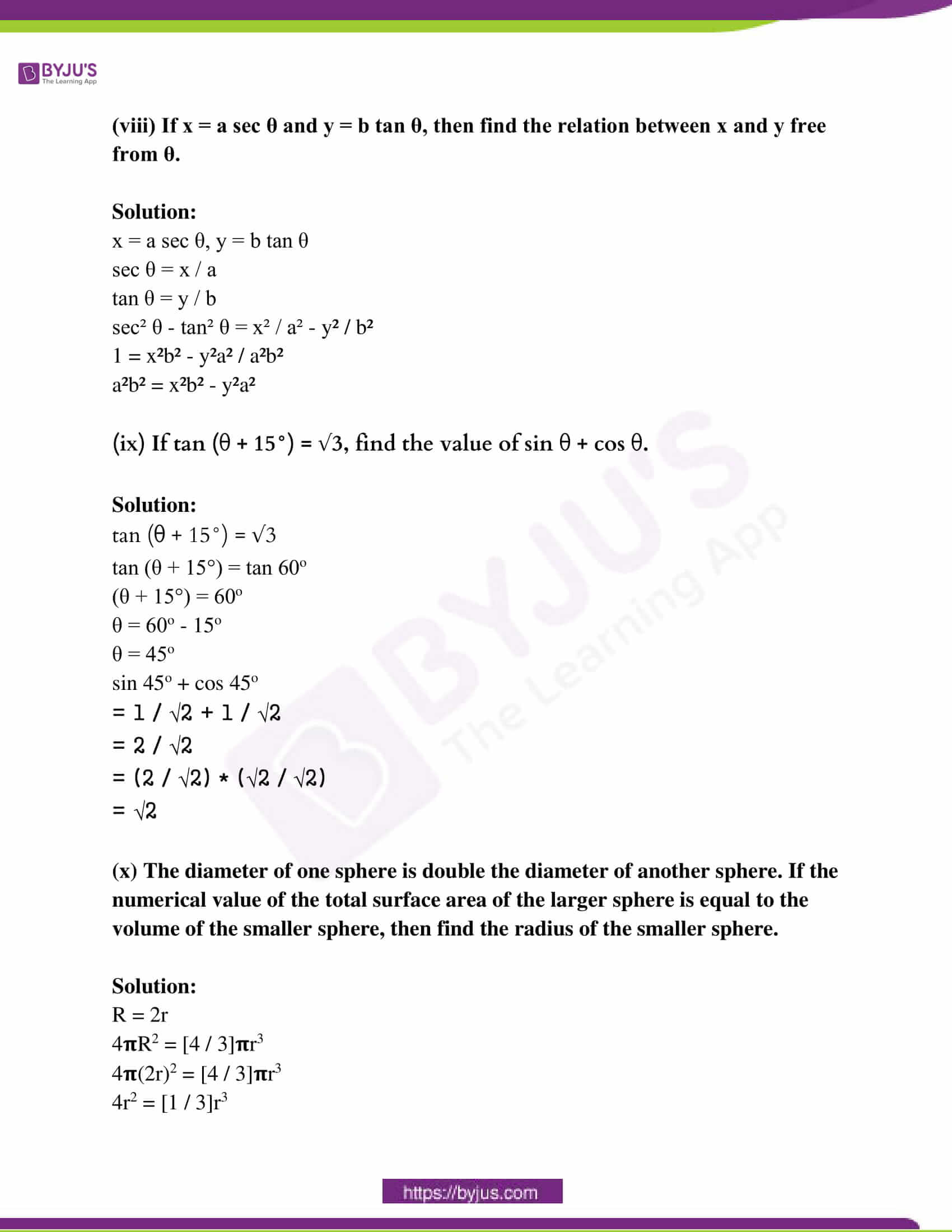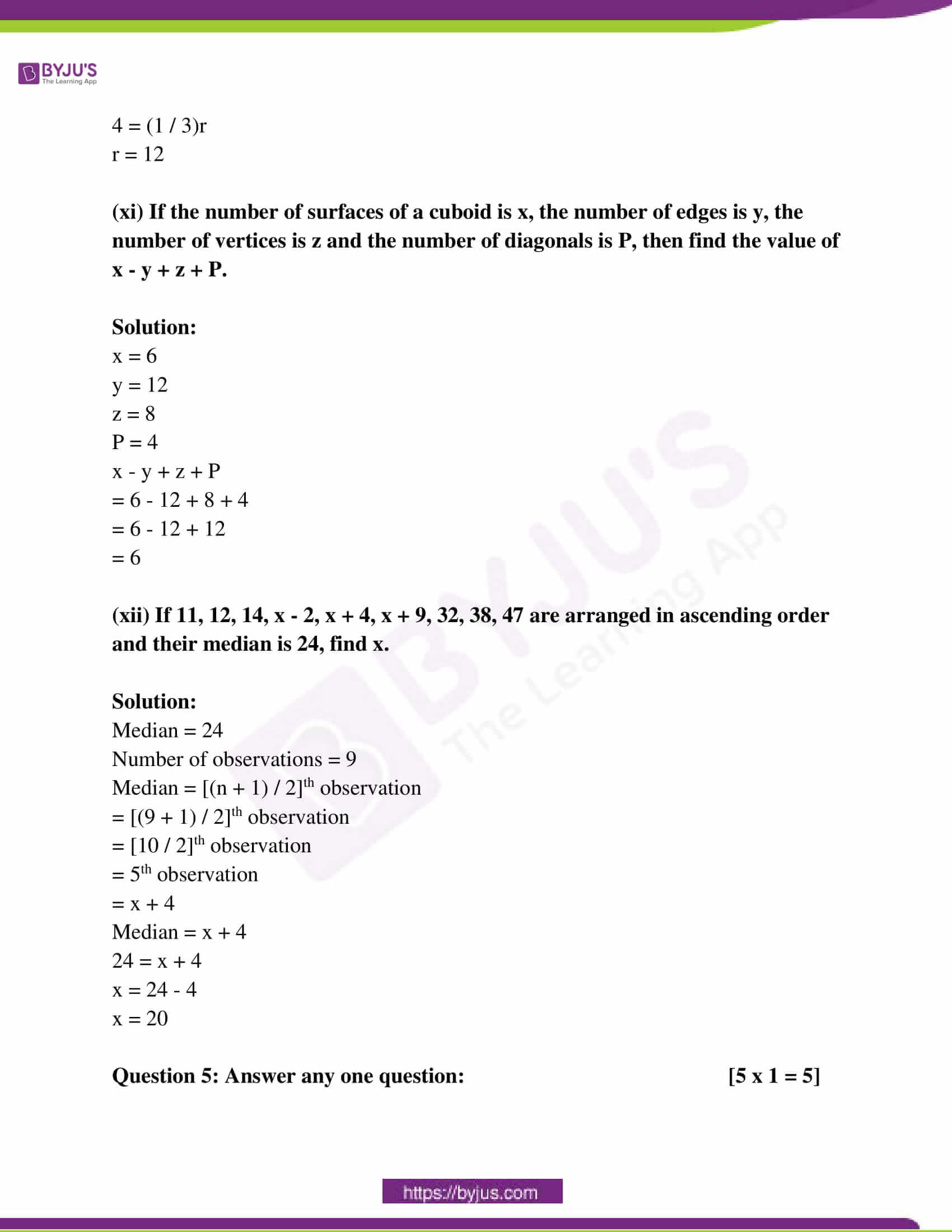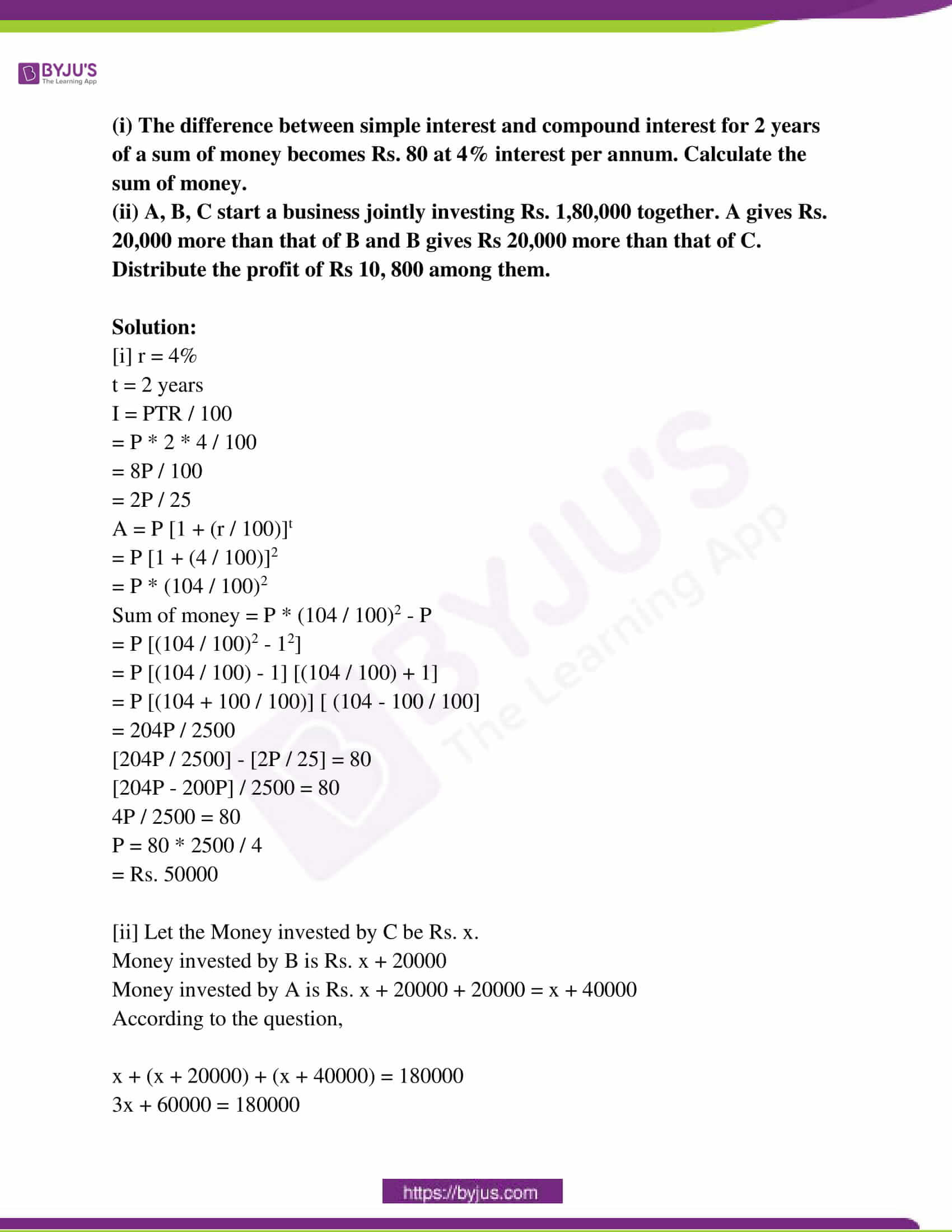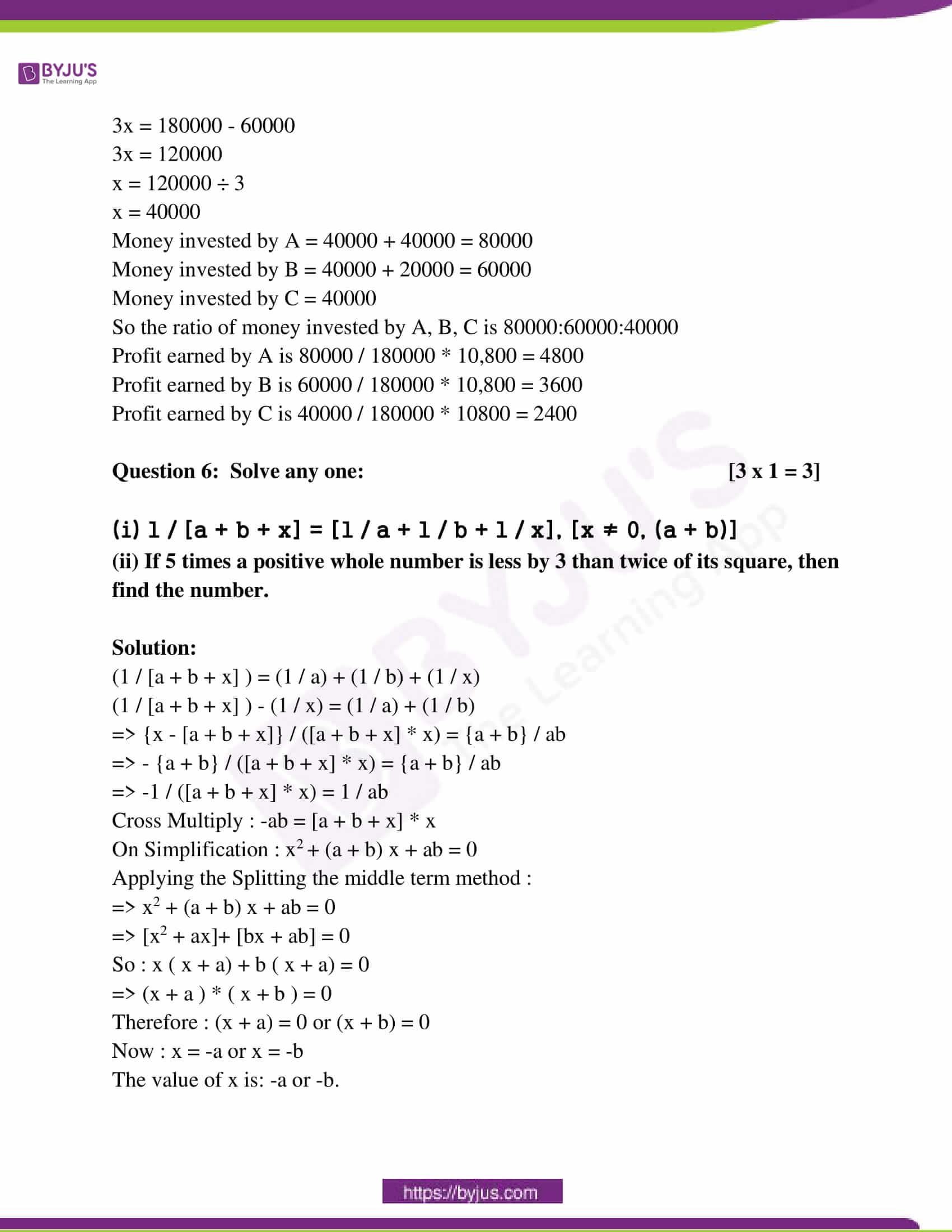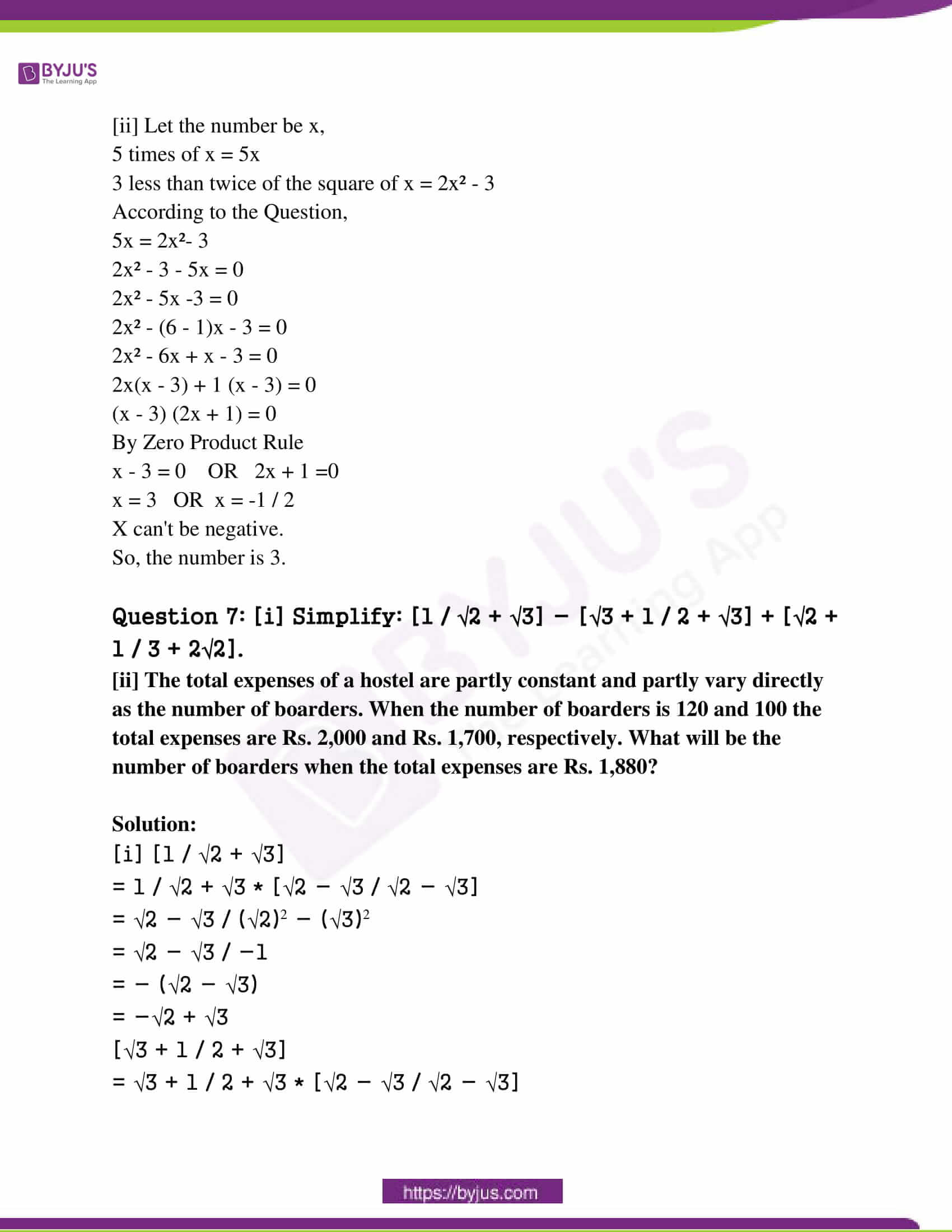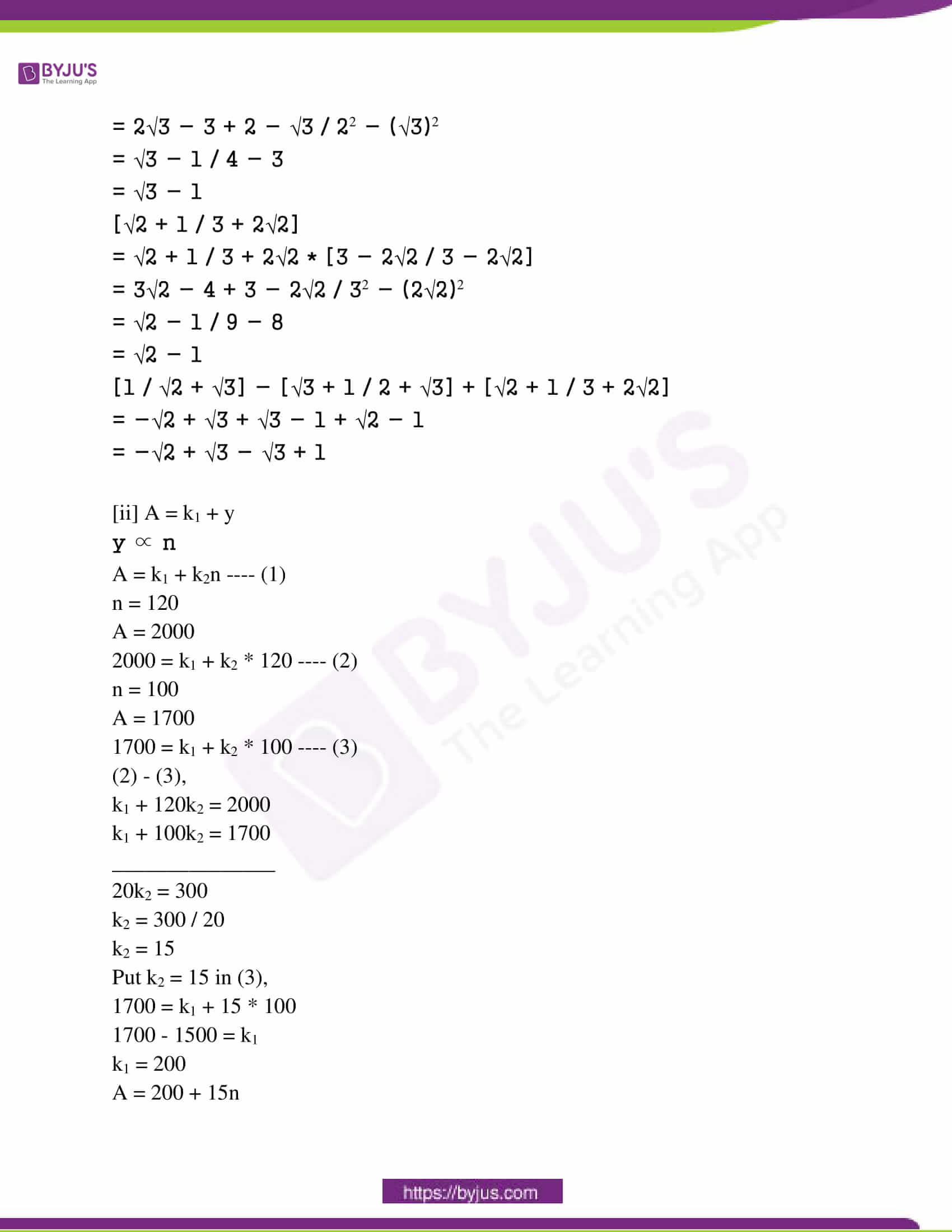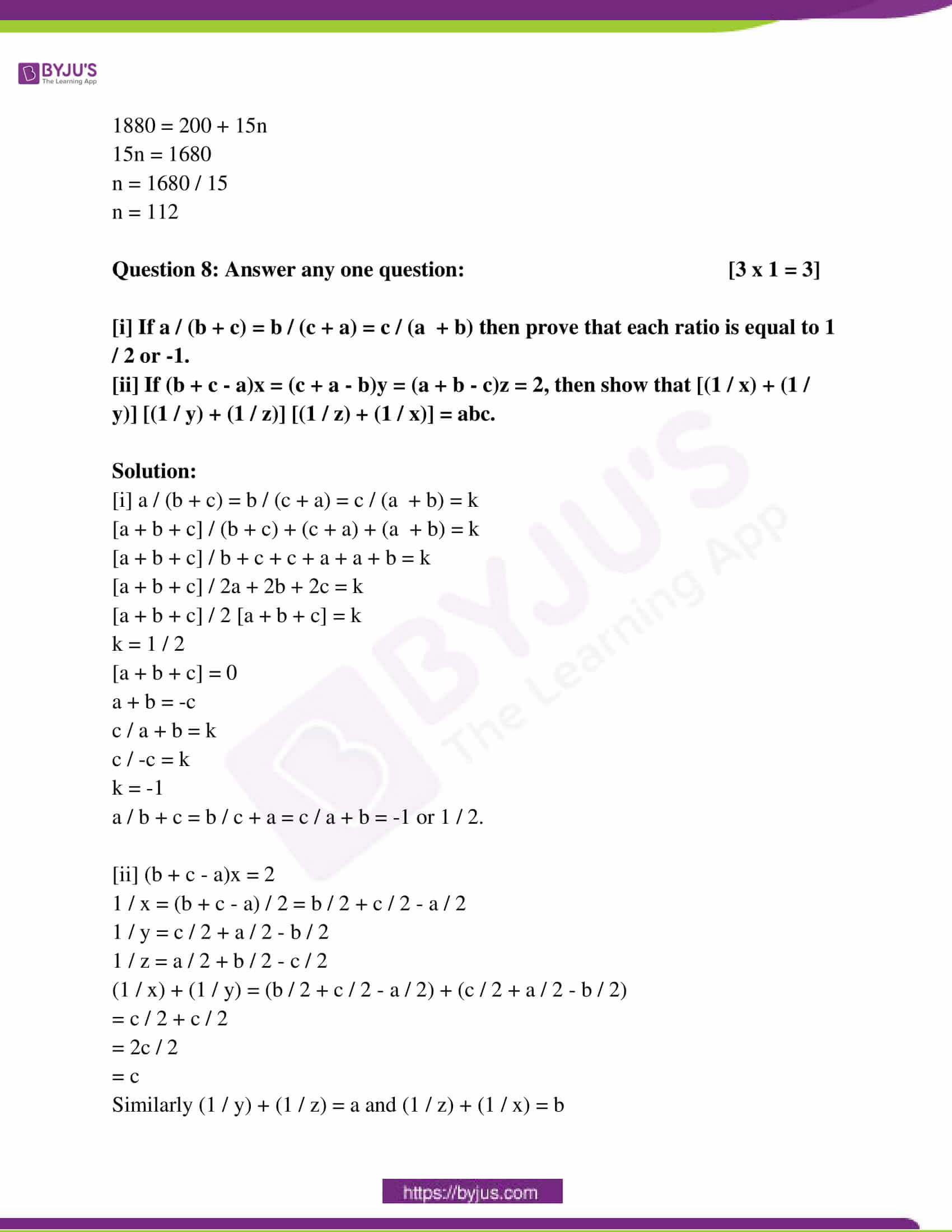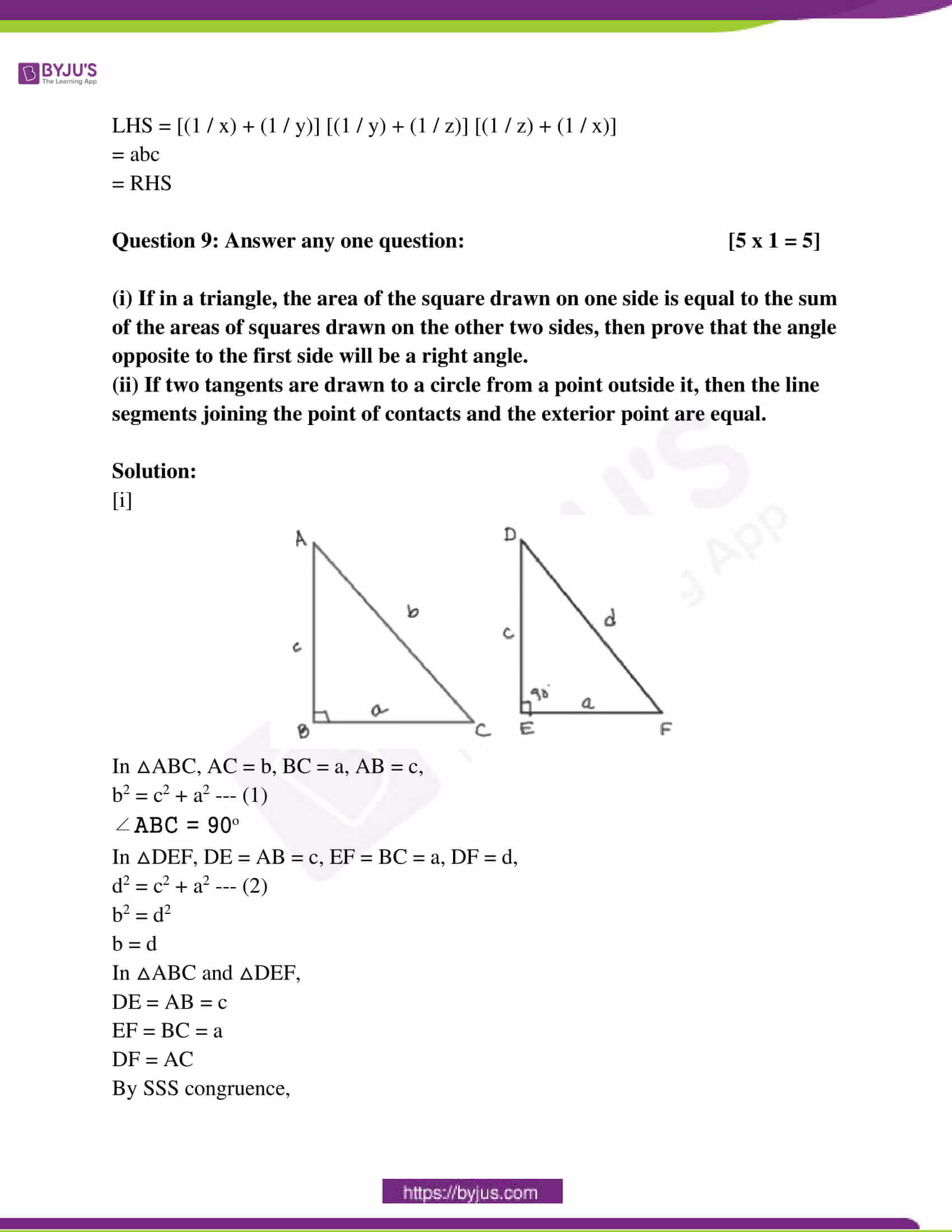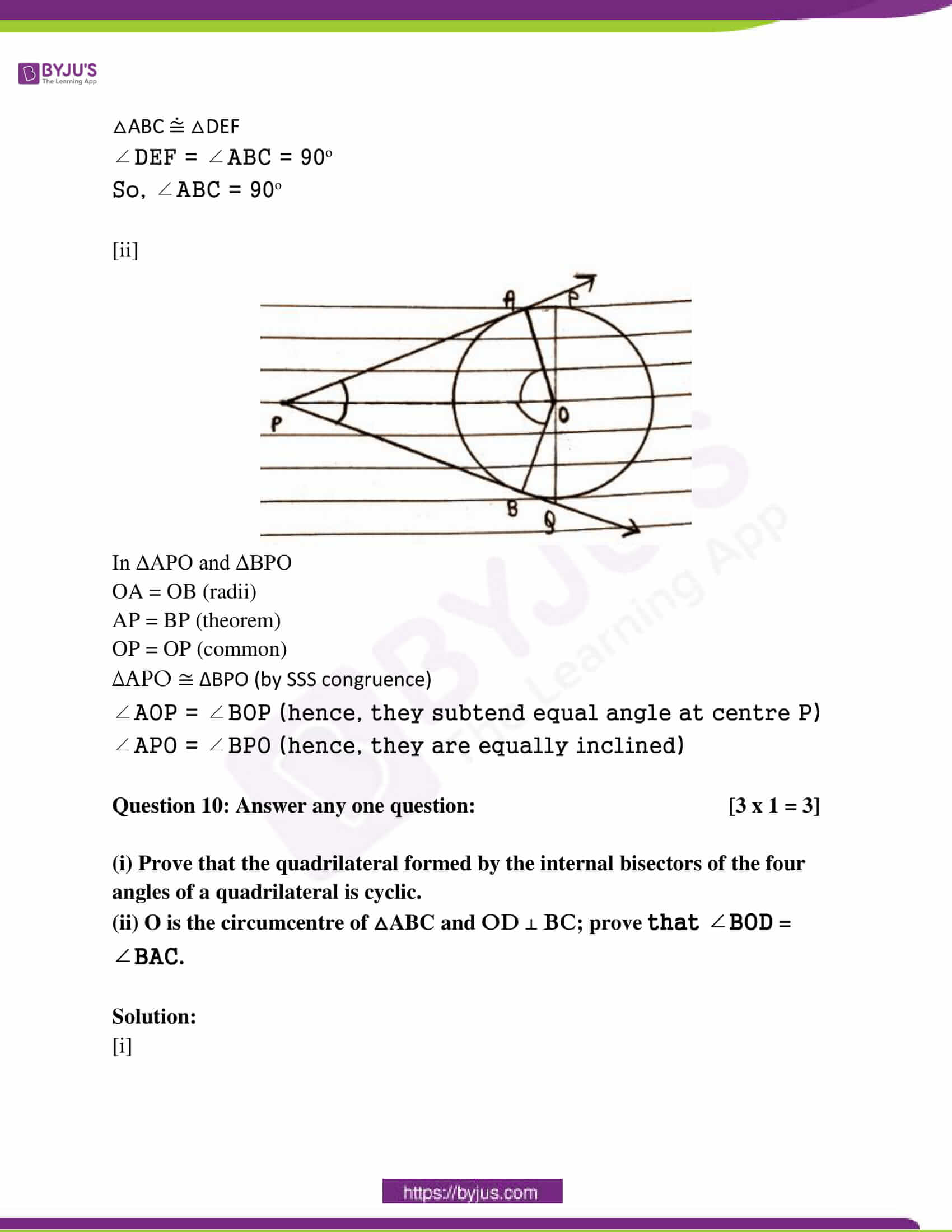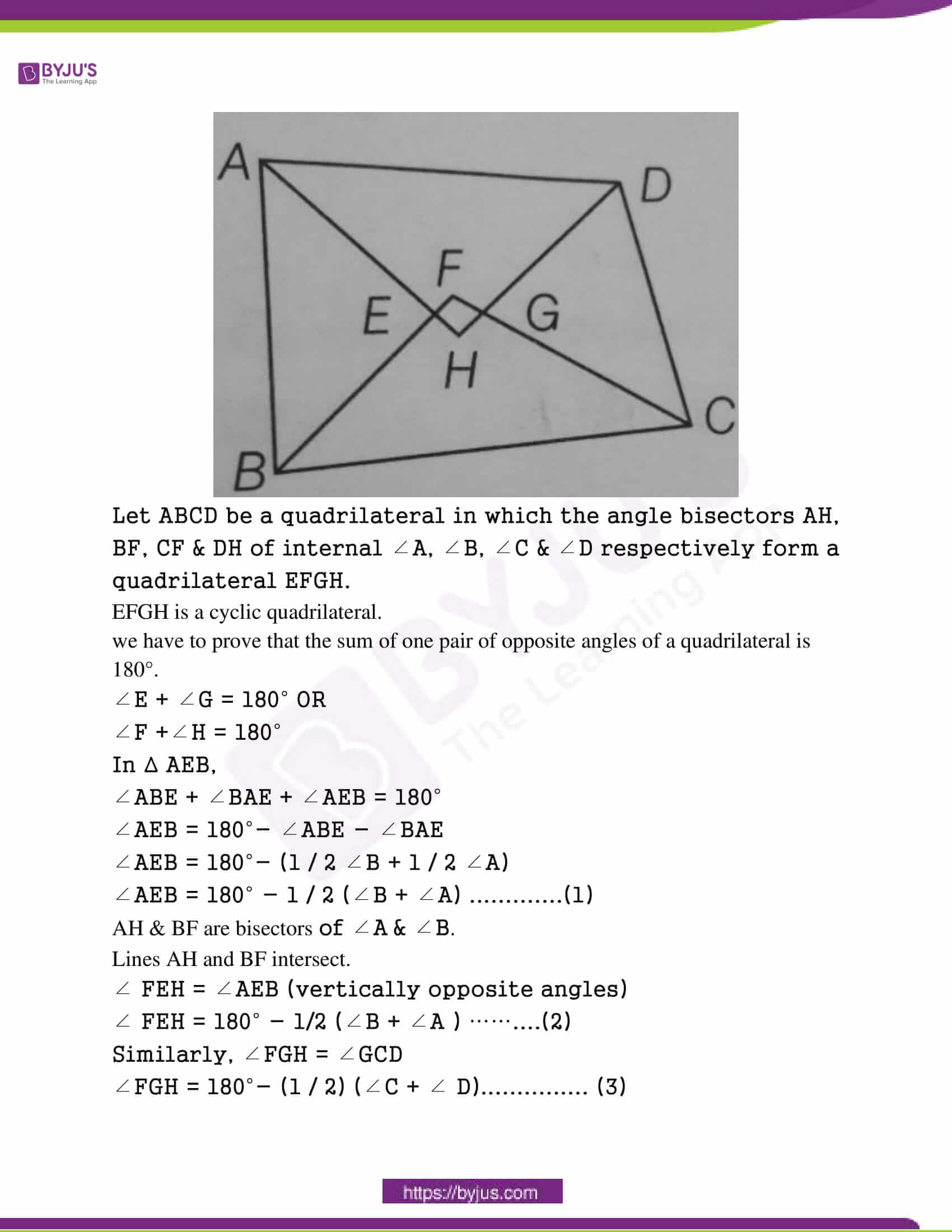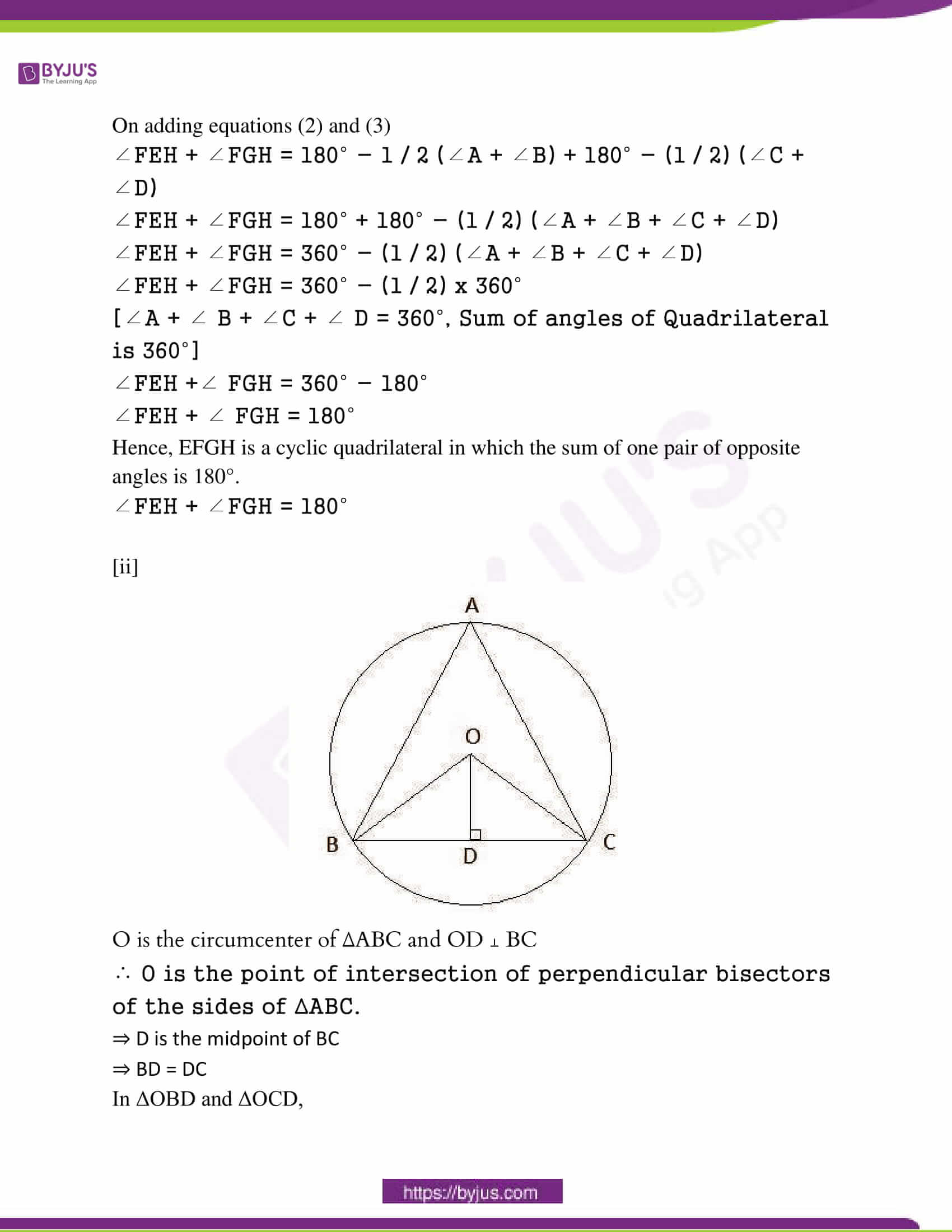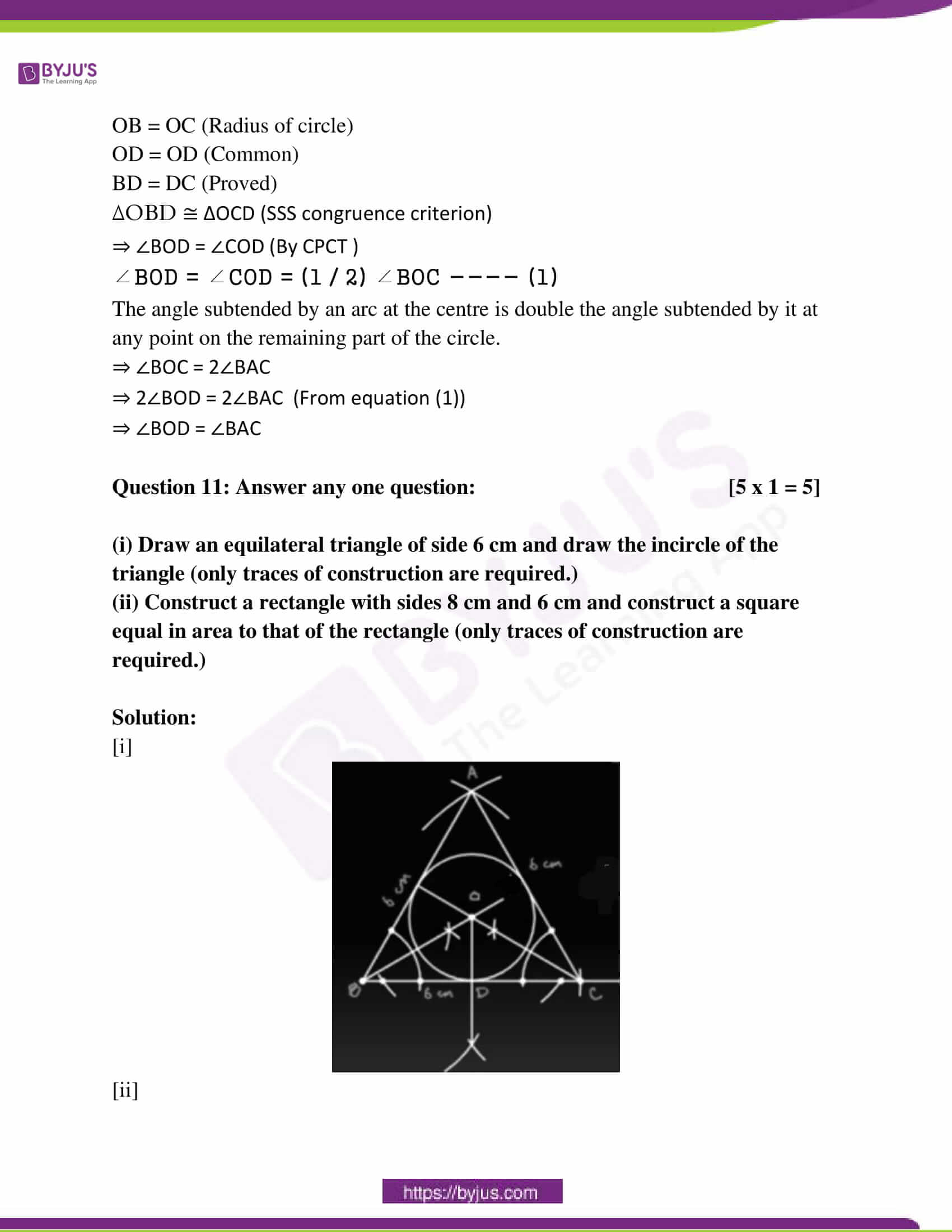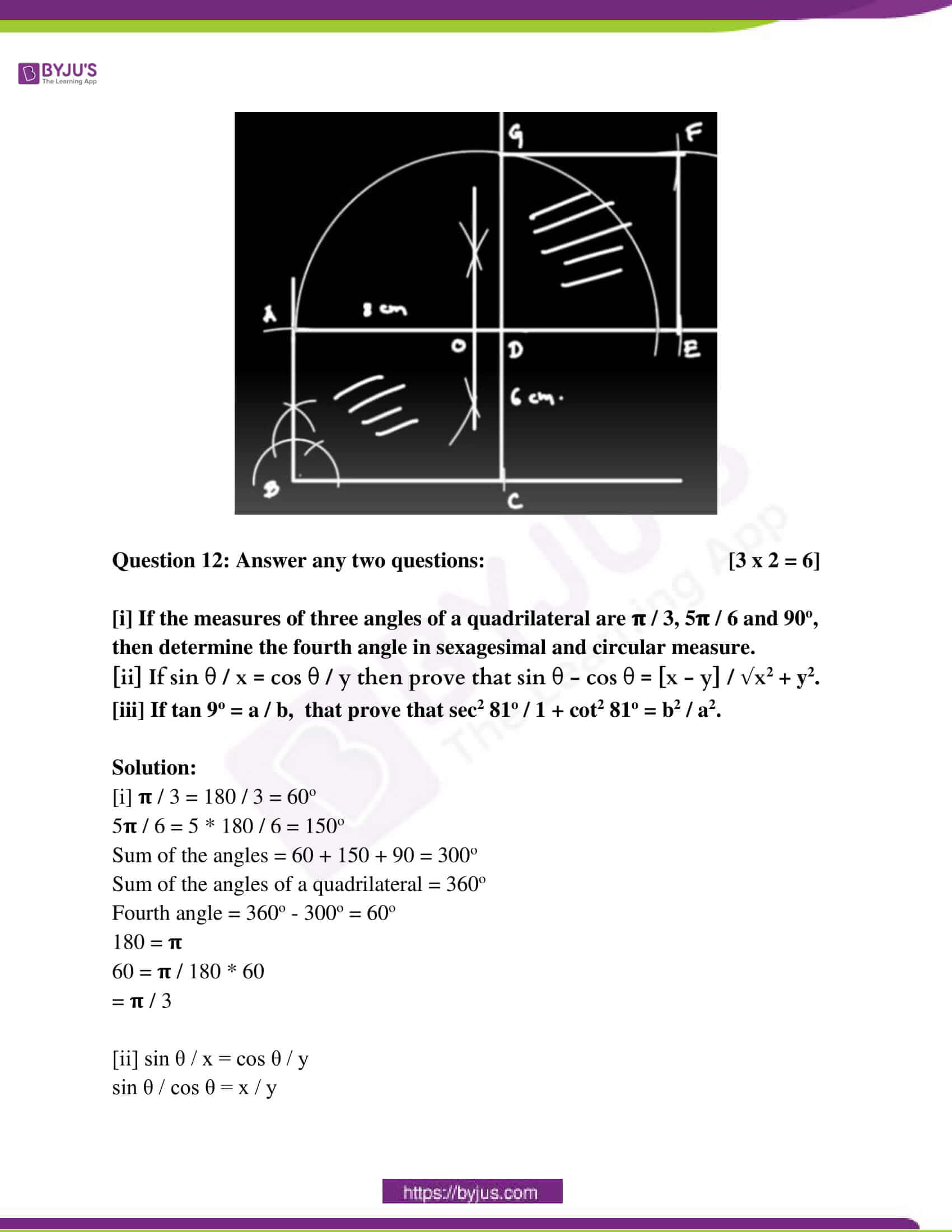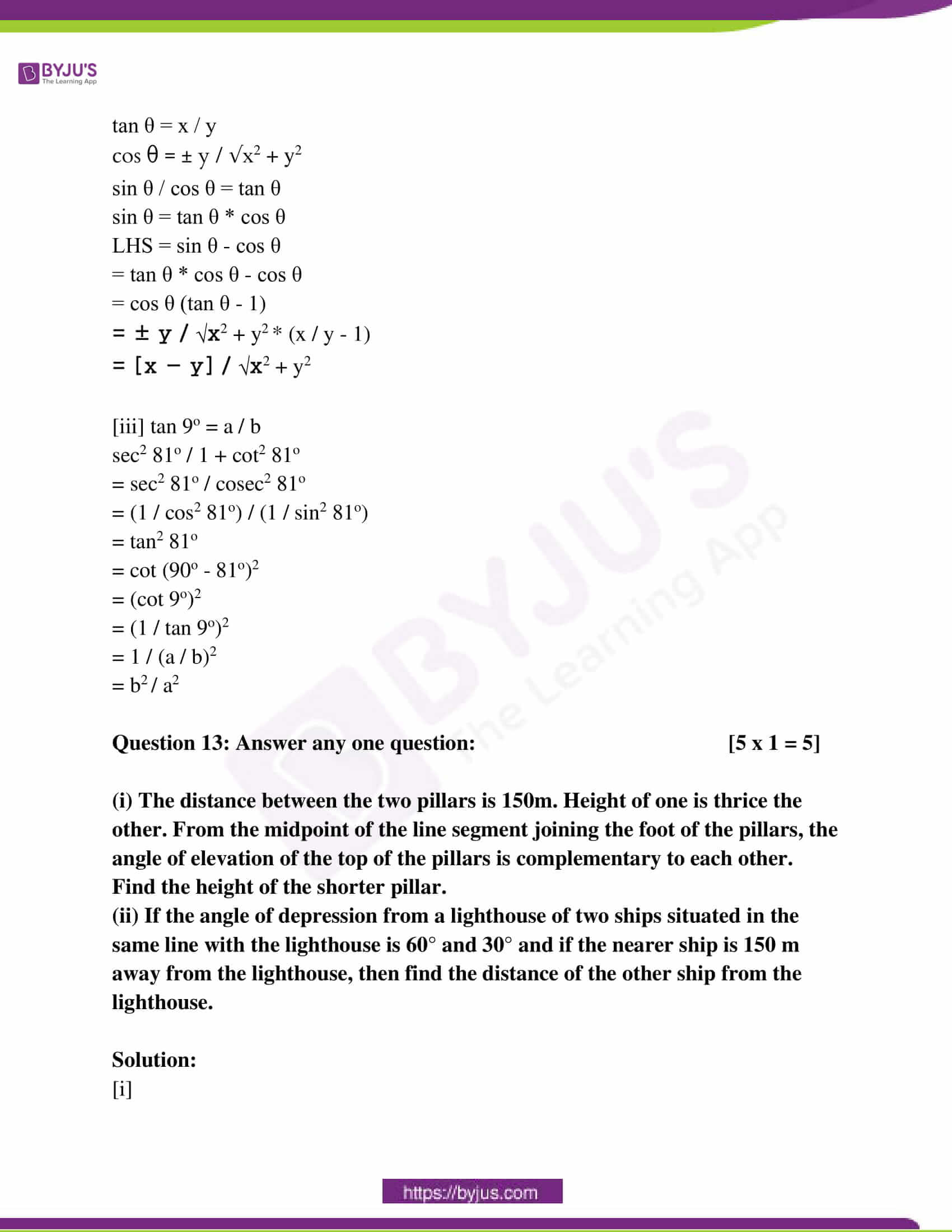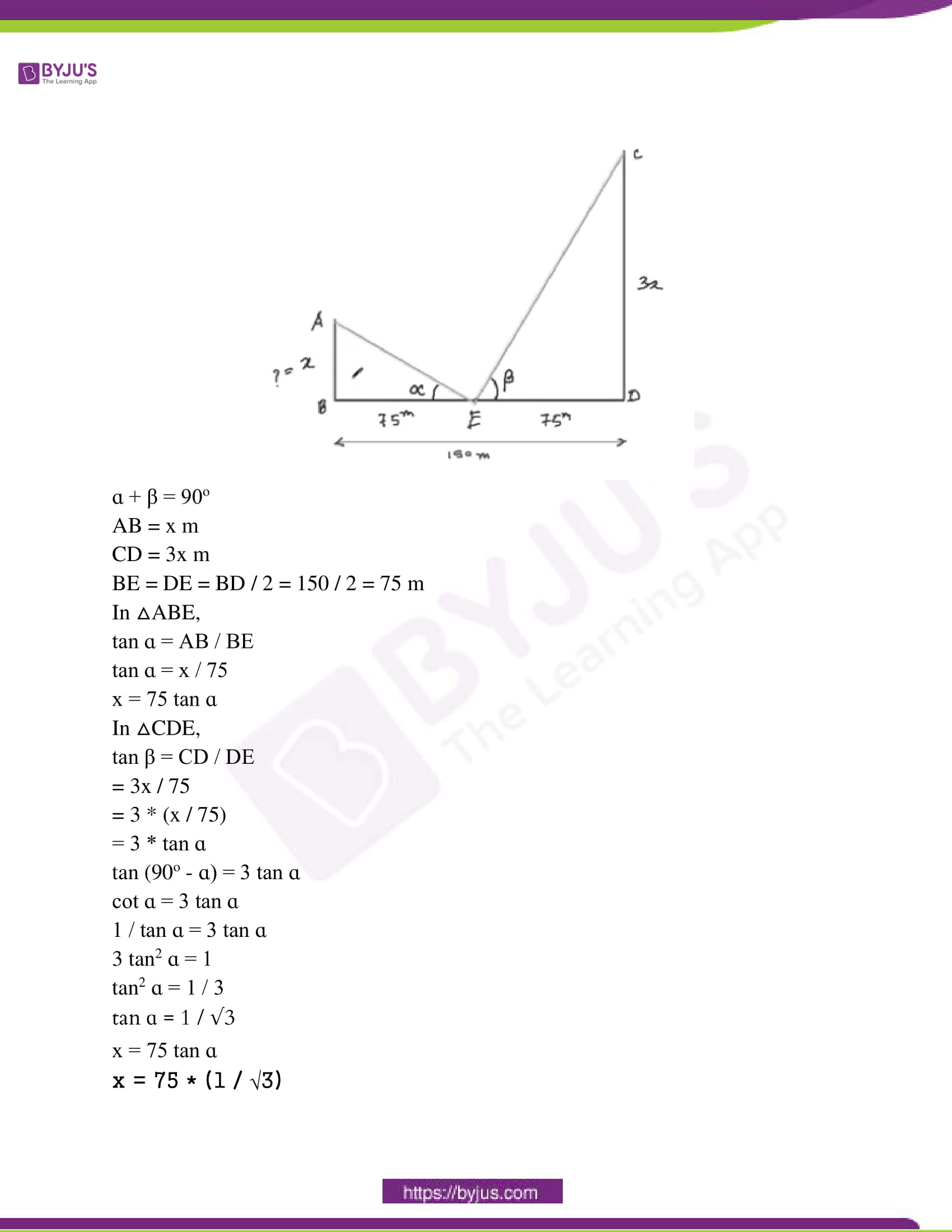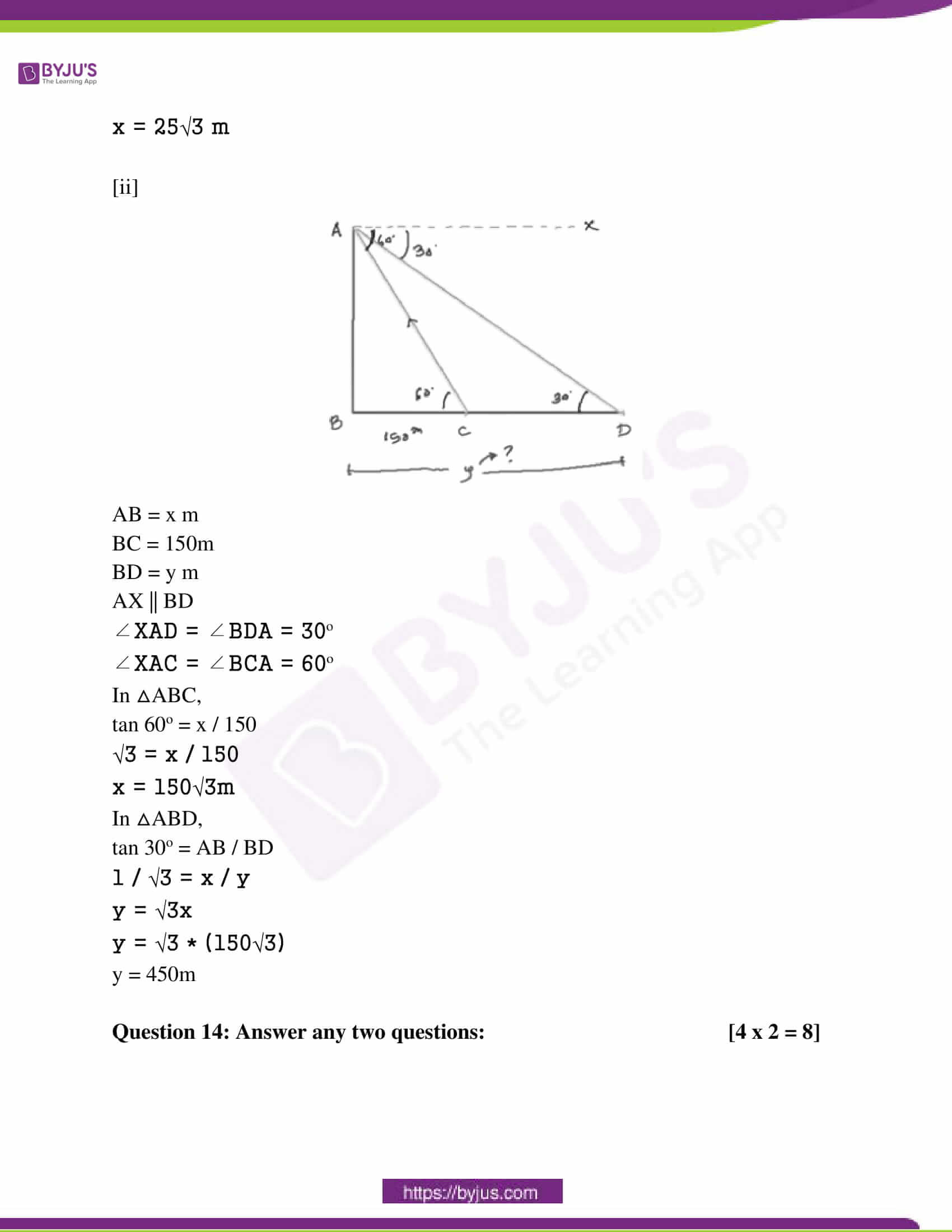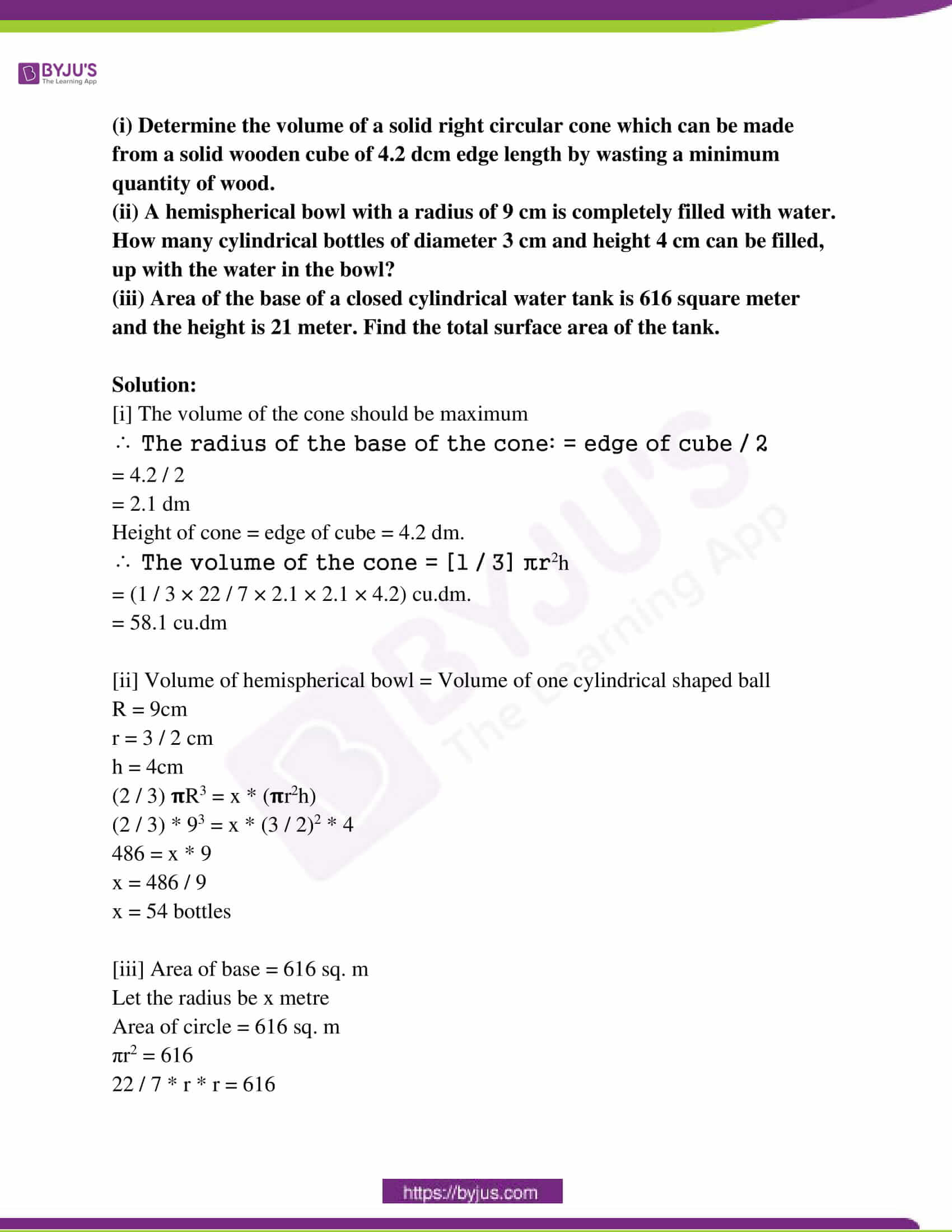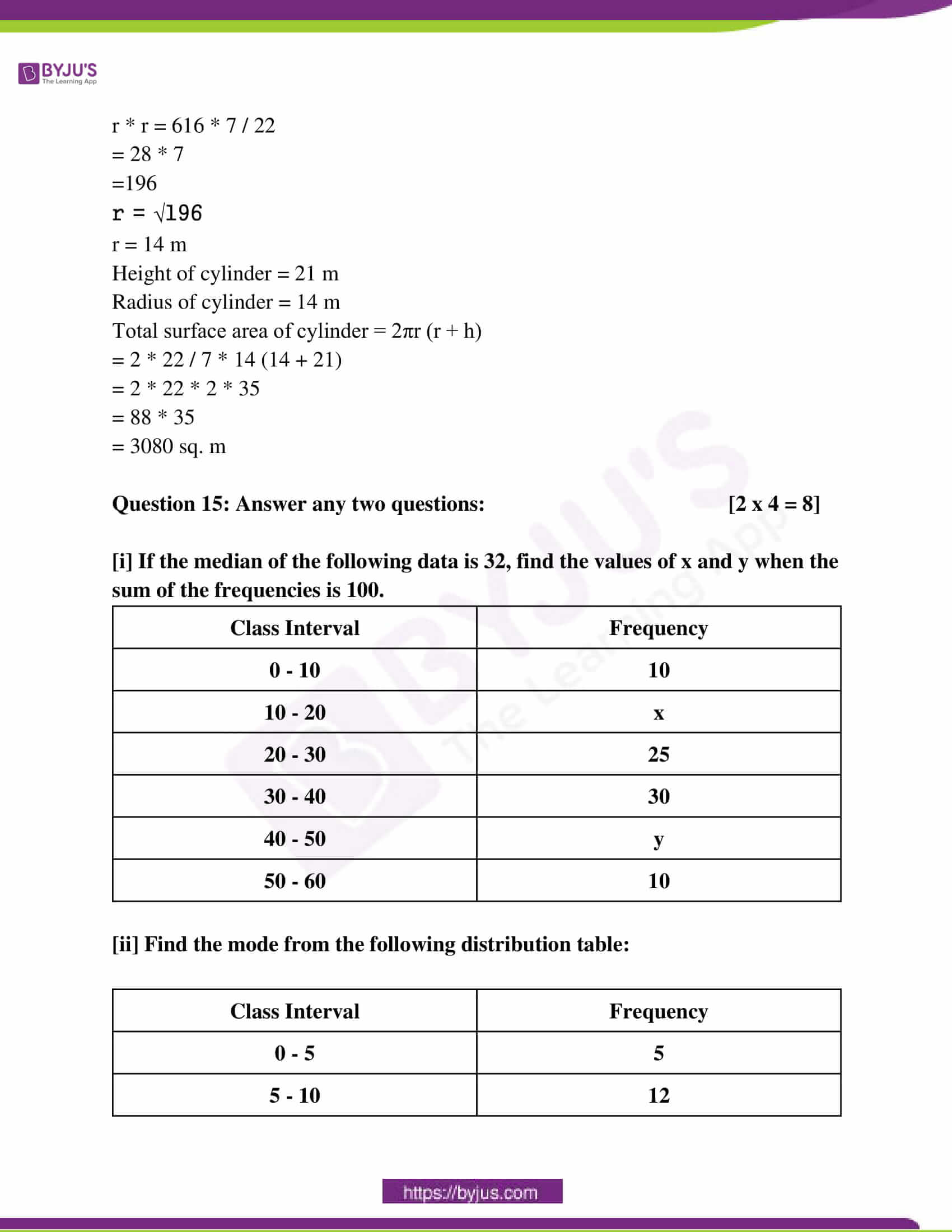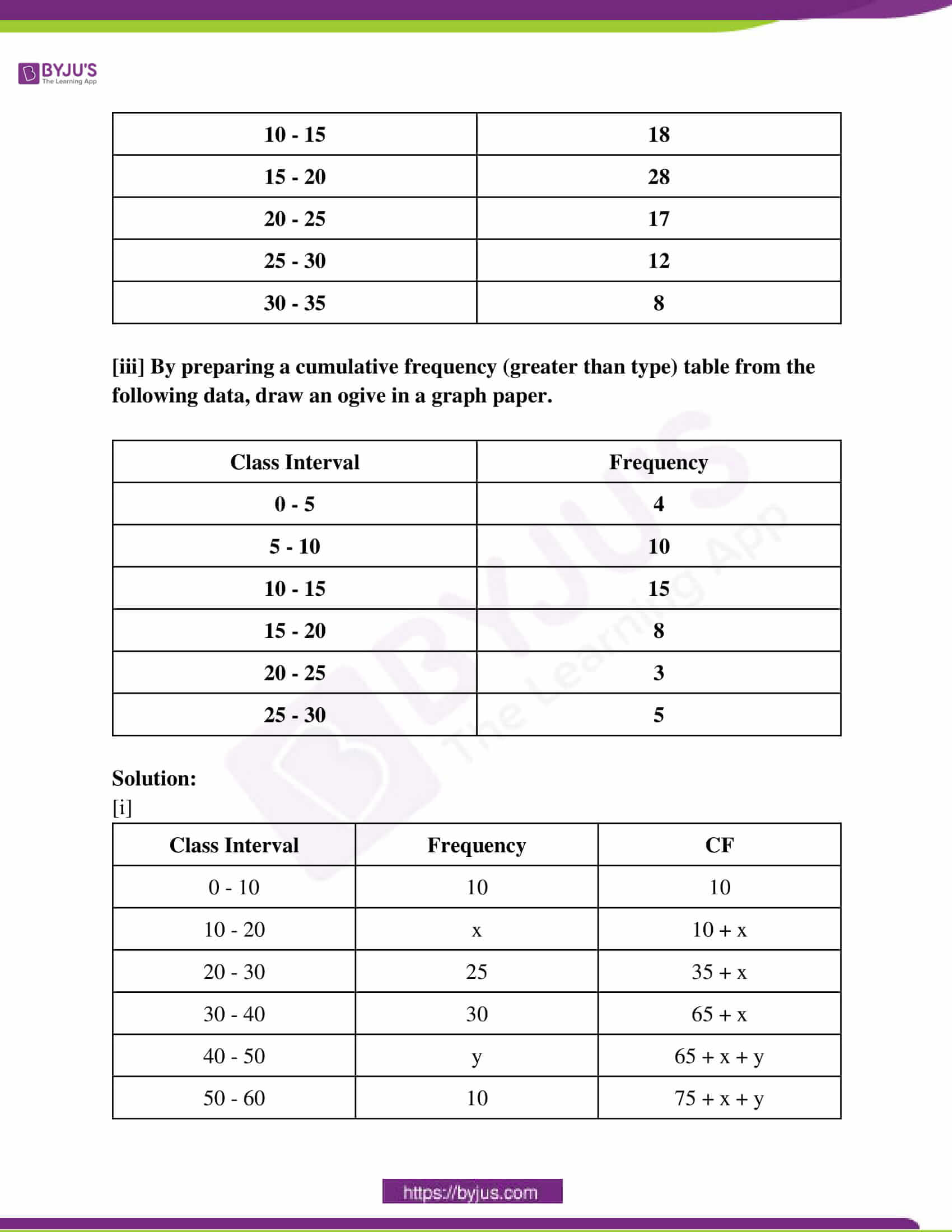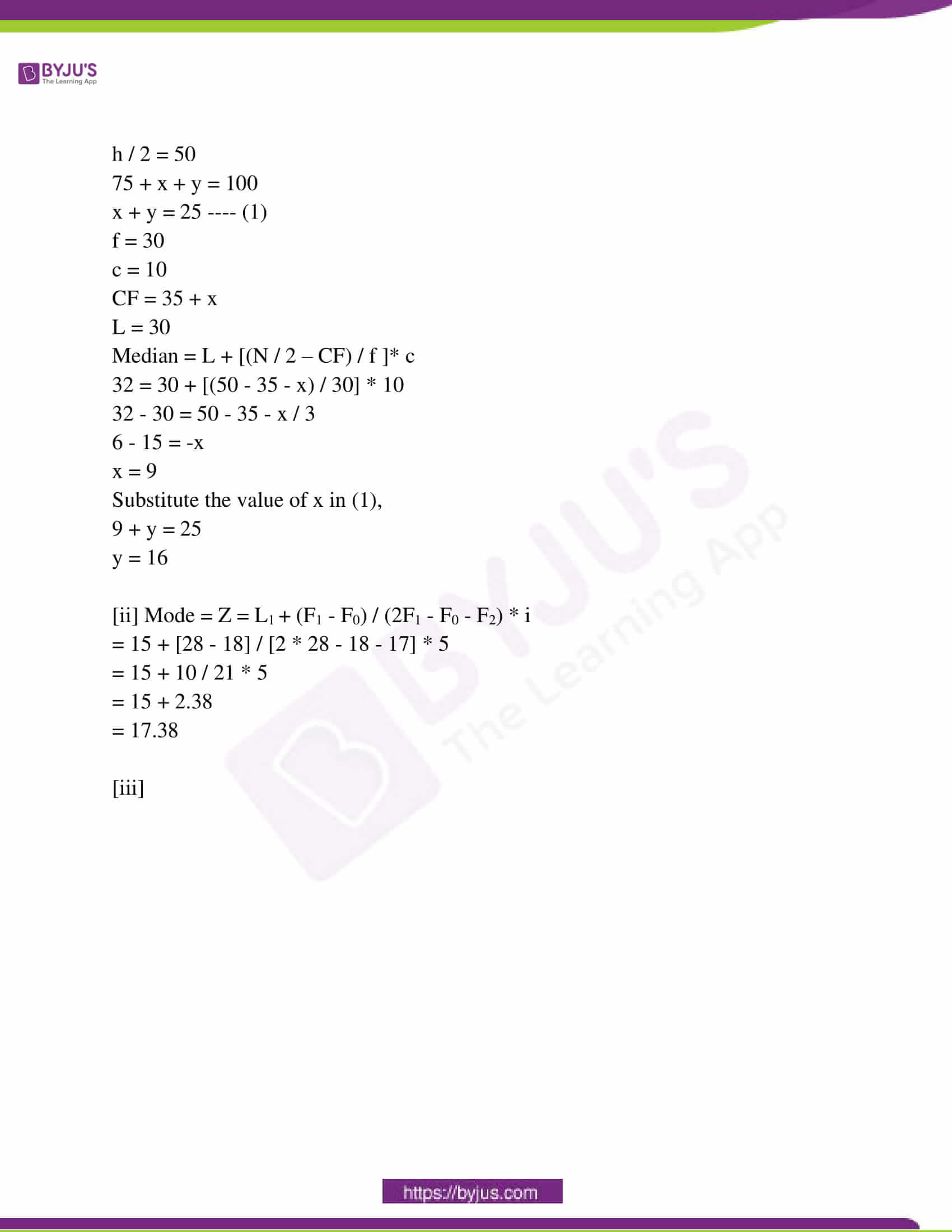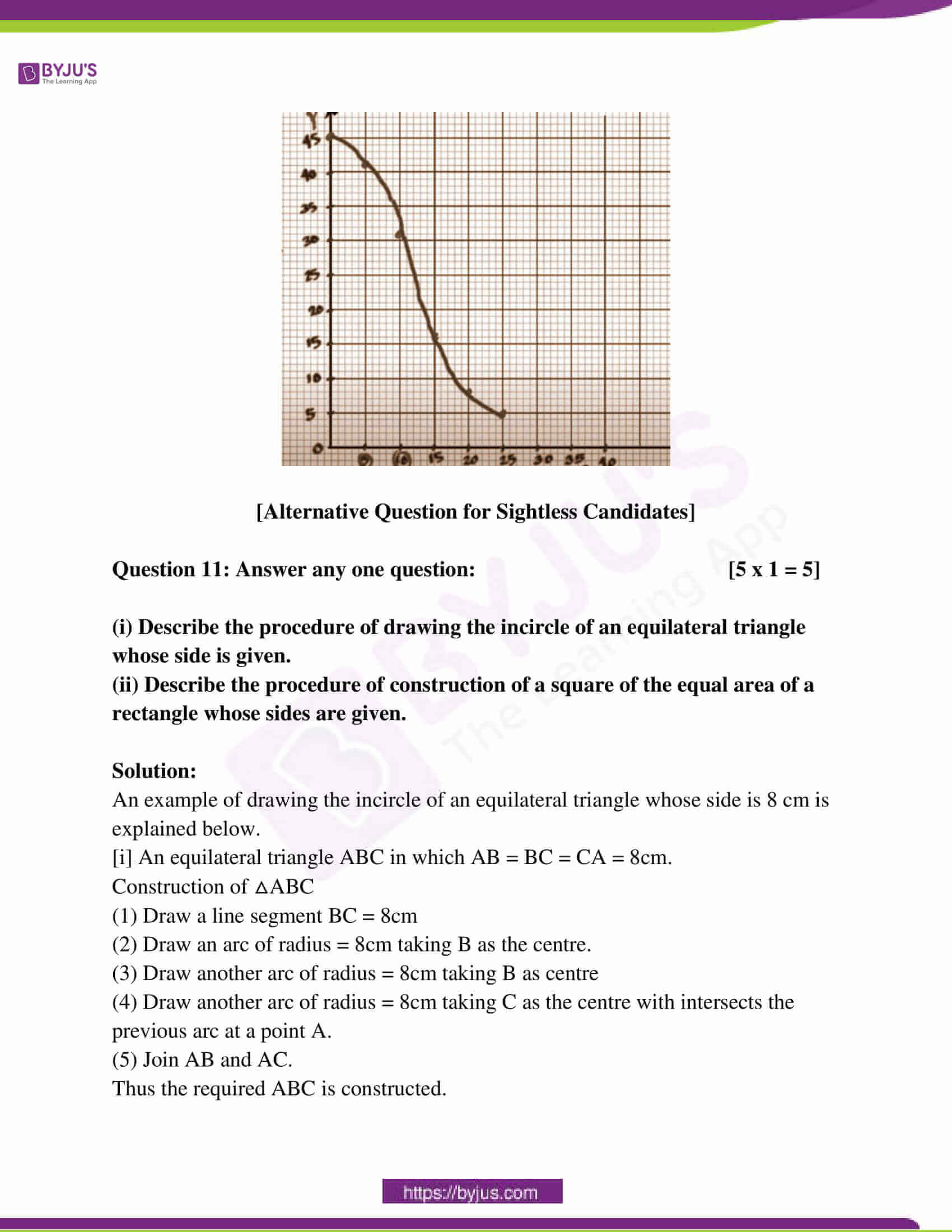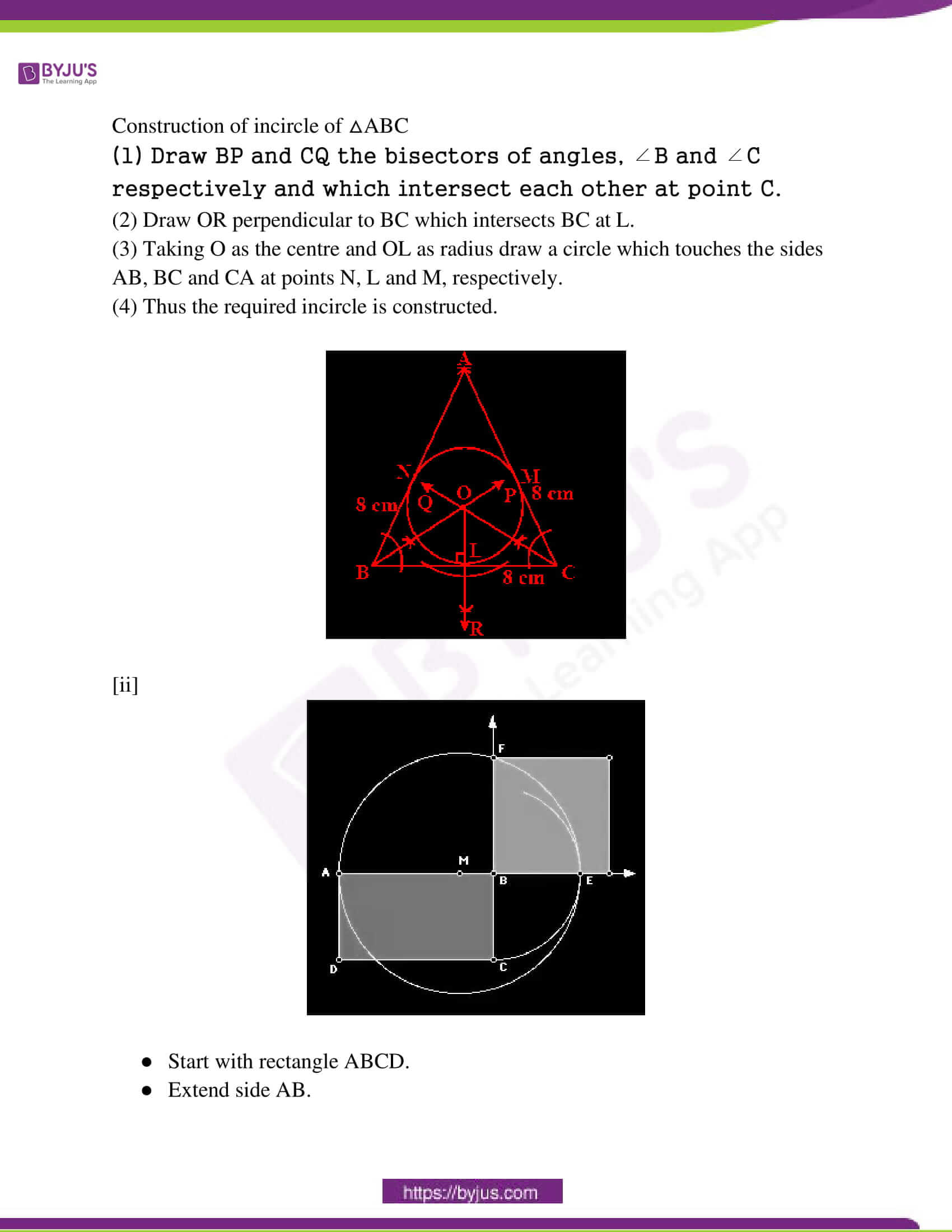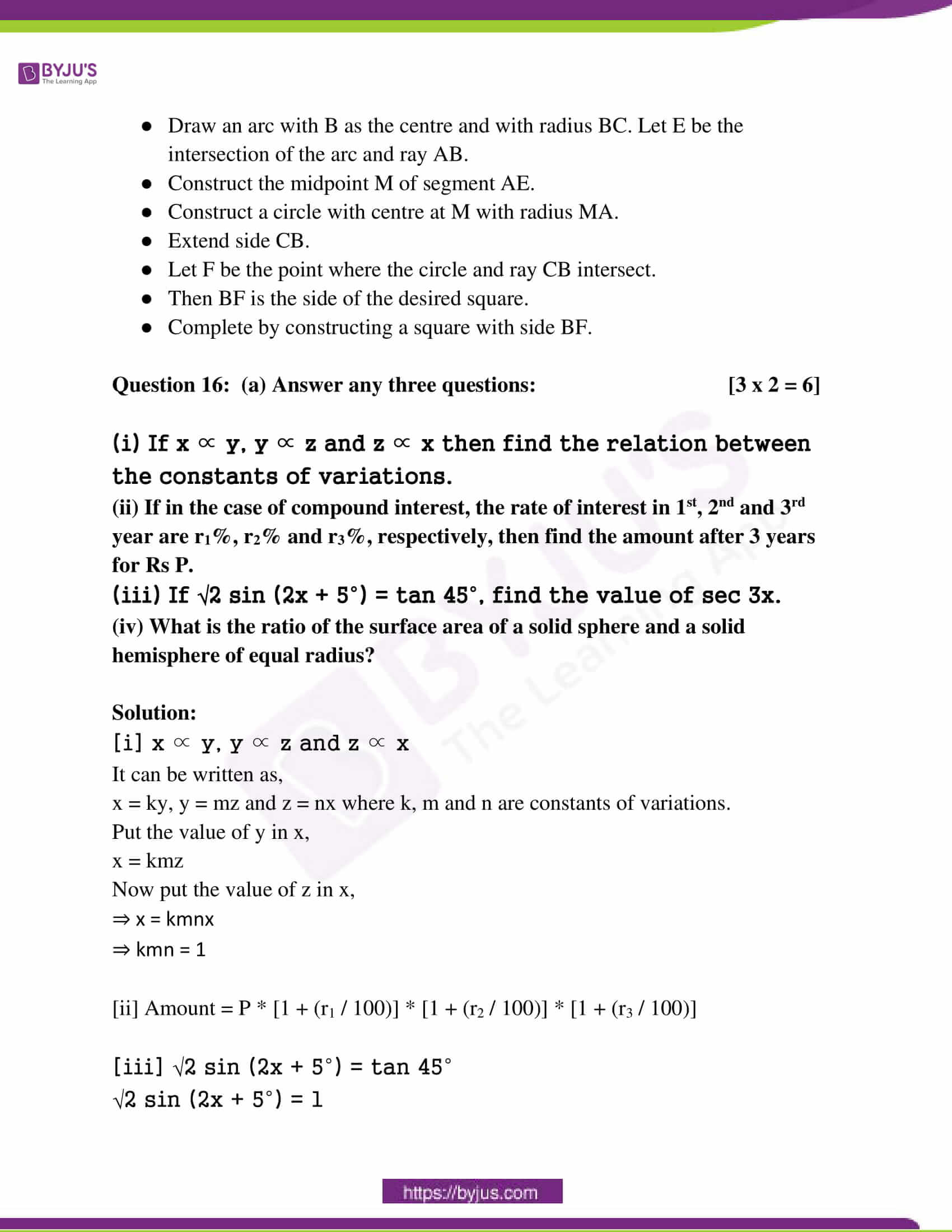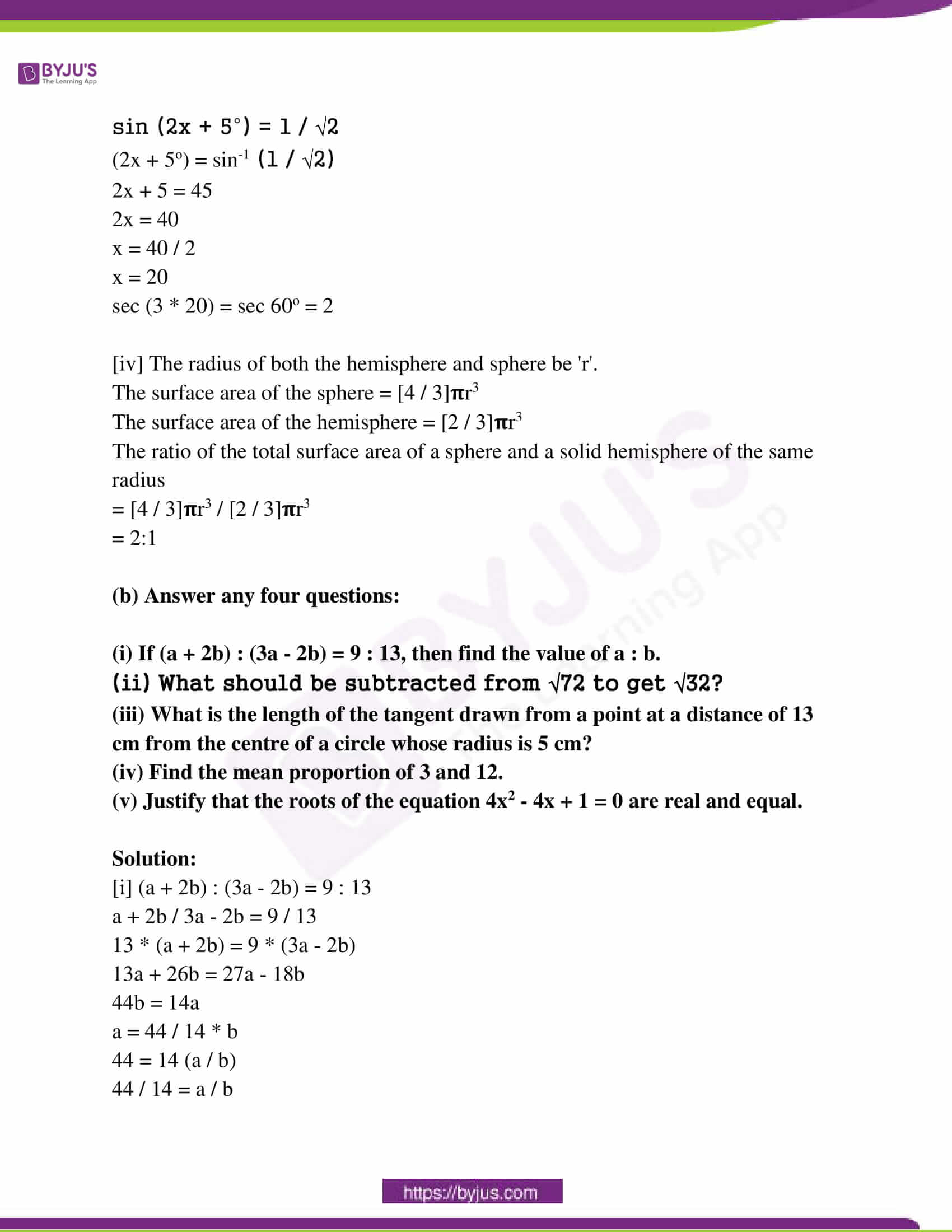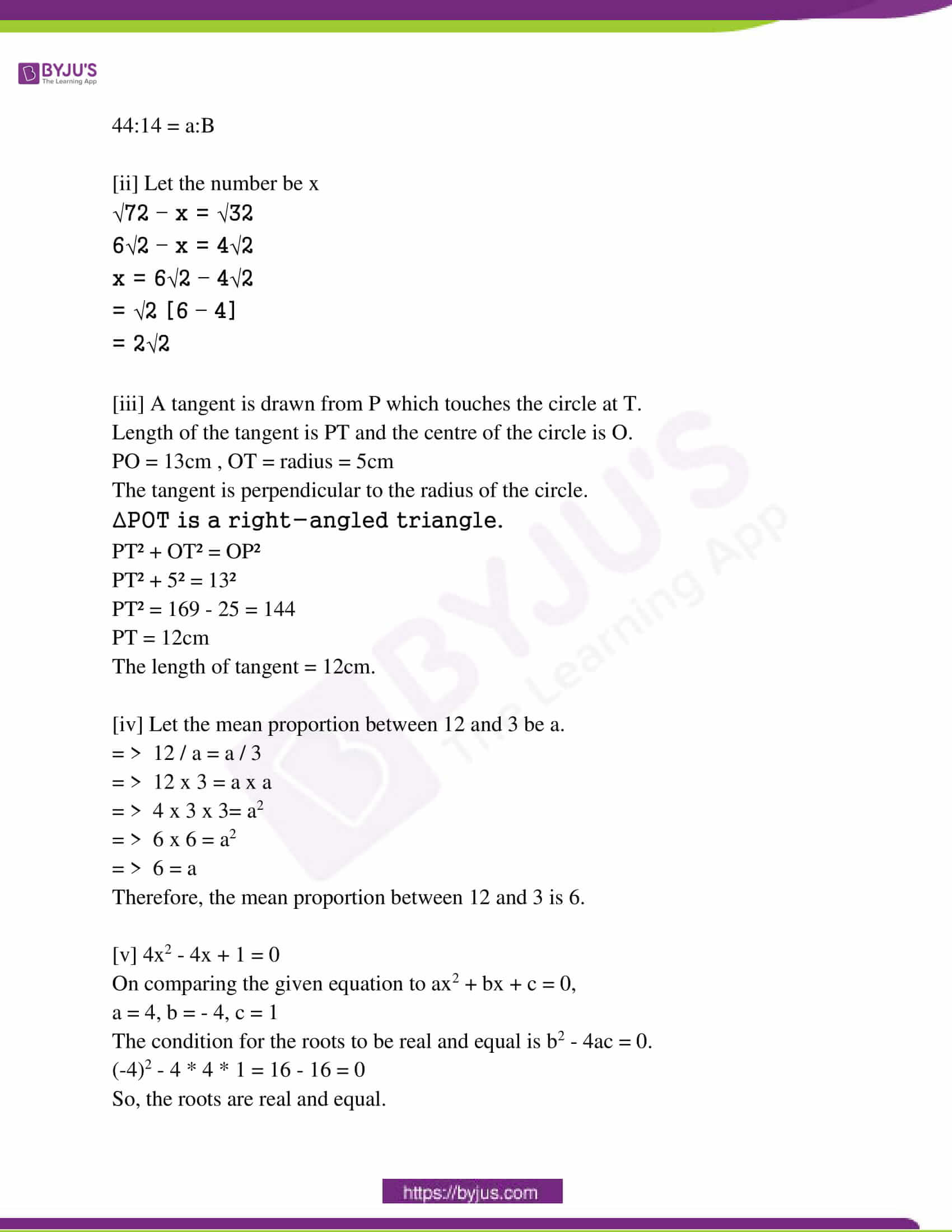Question 1: Choose the correct option in each case from the following questions: [1 x 6 = 6]

(i) If the ratio of principal and yearly amount be in the ratio 25:28, then the yearly rate of interest is

(a) 3% (b) 12% (c) 10 (5 / 7)% (d) 8%

R = SI * 100 / PT

= 28x – 25x * 100 / 25x * 1

= 3x / 25x

= 0.12 * 100

= 12%

(ii) Under what condition one root of the quadratic equation ax2 + bx + c = 0 is zero?

(a) a = 0 (b) b = 0 (c) c = 0 (d) None of these

(iii) The number of common tangents of two circles when they do not touch or intersect each other is

(a) 2 (b) 1 (c) 3 (d) 4

(iv) If sin θ = cos θ, then the value of 2θ will be

(a) 30° (b) 60° (c) 45° (d) 90°

sin θ = cos θ

sin θ = cos (90 – θ)

θ = 90o – θ

θ = 45o

2θ = 90o

(v) If each of radius of the base and height of a cone is doubled, then the volume of it will be

(a) 3 times (b) 4 times (c) 6 times (d) 8 times of the previous one

Let the height of the cone = h cm

The radius of the cone = r cm

Then the volume of the cone = (1 / 3)πr2h

If the height and radius are doubled then,

Height = 2h

Then new volume = (1 / 3)π * (2r)2* 2h

⇒ (1 / 3)π * 4r2 * 2h = 8 * (1 / 3) * πr2h​

Hence it is 8 times of the old volume.

(vi) The median of the numbers 2, 8, 2, 3, 8, 5, 9, 5, 6 is

(a) 8 (b) 6.5 (c) 5.5 (d) 5

Question 2: Fill in the blanks (any five): [1 x 5 = 5]

(i) At the same rate per cent per annum, the simple interest and compound interest of the same principal are the same in _____ year. 

(ii) If in a quadratic equation ax2 + bx + c = 0 (a ≠ 0) b2 = 4ac, then the roots of the equation will be real and _____. [equal]

(iii) If the length of the sides of two triangles are in proportion, then two triangles are ______. [similar]

(iv) If cos2 θ – sin2 θ = 1 / x (x > 1), then cos4 θ – sin4 θ = ______ . [1 / x]

(v) The numbers of the plane surface of a solid hemisphere are ______. 

(vi) If the mean of x1, x2, x3, x4 …… xn be the mean, then the mean of Kx1, Kx2, Kx3…….. Kxn is _____ (K ≠ 0). [k * (mean)]

Question 3: Write True or False (any five): [1 x 5 = 5]

(i) A starts a business with Rs. 10,000 and B give Rs. 20,000 after 6 months. At the end of the year, their profit will be equal. [True]

(ii) If x + 2√3, the value of x + 1 / x is 2√3. [False]

(iii) If two circles of radii 7 cm and 3 cm touch each other. externally, then the distance between their centres will be 4 cm. [False]

(iv) If 0° < θ < 90°, then sin (sin θ) > sin2θ. [True]

(v) If the total surface area of a hemisphere is 36𝛑 sq. cm., then its radius will be 3 cm. [False]

(vi) If the perpendicular drawn on the x-axis from the point of intersection of both ogive, the abscissa of the point of intersection of this perpendicular with the x-axis will be the median. [True]

Question 4: Answer the following questions (any ten): [2 x 10 = 20]

(i) A sum of money is doubled in 8 years at r% rate of compound interest per annum. At the same rate in how many years will it be four times the sum?

Solution:

A = P [1 + (r / 100)]t

2P = P [1 + (r / 100)]8

[1 + (r / 100)]8 = 2

1 + (r / 100) = 21/8

4P = P [1 + (r / 100)]t

[1 + (r / 100)]t = 4

(21/8))t = 4

21/8)t = 22

2t/8 = 22

t / 8 = 2

t = 16 years

(ii) A invests 1½ times more than B invests in a business. At the end of the year, B receives Rs. 1,500 as profit. How much profit A will get at the end of that year?

Solution:

A = 1½ * B

= 3 / 2 * B

= 3 / 2 * 1500

= Rs. 2250

(iii) Without solving, find the values of ‘p’ for which the equation x2 + (p – 3)x + p = 0 has real and equal roots.

Solution:

x2 + (p – 3) x + p = 0

Here, a = 1, b = (p – 3), c = p

Since, the roots are real and equal, D = 0

b2 – 4ac = 0

(p – 3)2 – 4(1) (p) = 0

p2 + 9 – 6p – 4p = 0

p2 – 10p + 9 = 0

(p – 1)(p – 9) = 0

p = 1 or p = 9

(iv) If x ∝ yz and y ∝ zx show that z is a non-zero constant.

Solution:

x ∝ yz

x = k1yz

y ∝ zx

y = k2zx

y = k2z (k1yz)

y = k2k1yz2

k2k1z2 = 1

z2 = 1 / k2k1

z = ± √1 / k2k1

So, z is a non zero constant.

(v) The perimeter of two similar triangles is 20 cm and 16 cm respectively. If the length of one side of the first triangle is 9 cm, then find the length of the corresponding side of the second triangle.

Solution:

Let the side of the second triangle be x.

In similar triangles,

Ratios of perimeters = ratios of lengths of sides – (1)

The ratio of perimeter = 20:16 = 5:4 – (2)

The ratio of lengths of sides = 9:x – (3)

From (1), (2) and (3),

5:4 = 9:x

5 / 4 = 9 / x

5x = 36

x = 36 / 5

x = 7.2 cm

(vi) In ABC, ∠ABC = 90o, AB = 5 cm, BC = 12 cm. Find the length of the circumradius of ABC.

Solution:

ABC is a triangle, in which ∠B = 90°, AB = 12 cm , BC = 5 cm

BC is the base of the ΔABC, AB is the perpendicular of ΔABC and AC is the hypotenuse of ΔABC.

By Pythagoras theorem,

H² = P² + B²

AC² = 12² + 5²

AC² = 144 + 25

AC² = 169

AC = √169

AC = 13

So, the hypotenuse is 13 cm

The hypotenuse is the circumradius of the triangle.

(vii) In ABC, If AB = (2a – 1) cm, AC = 2√2a cm and BC = (2a + 1) cm, then write down the value of ∠ABC.

Solution: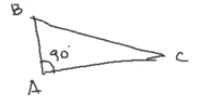AB2 + AC2 = BC2

AB2 = (2a – 1)2

= 4a2 – 4a + 1

AC2 = (2√2a)2 = 8a

AB2 + AC2 = 4a2 – 4a + 1 + 8a = 4a2 + 4a + 1

BC2 = (2a + 1)2

= 4a2 + 4a + 1

Hence, AB2 + AC2 = BC2.

So, ∠ABC = 90o

(viii) If x = a sec θ and y = b tan θ, then find the relation between x and y free from θ.

Solution:

x = a sec θ, y = b tan θ

sec θ = x / a

tan θ = y / b

sec² θ – tan² θ = x² / a² – y² / b²

1 = x²b² – y²a² / a²b²

a²b² = x²b² – y²a²

(ix) If tan (θ + 15°) = √3, find the value of sin θ + cos θ.

Solution:

tan (θ + 15°) = √3

tan (θ + 15°) = tan 60o

(θ + 15°) = 60o

θ = 60o – 15o

θ = 45o

sin 45o + cos 45o

= 1 / √2 + 1 / √2

= 2 / √2

= (2 / √2) * (√2 / √2)

= √2

(x) The diameter of one sphere is double the diameter of another sphere. If the numerical value of the total surface area of the larger sphere is equal to the volume of the smaller sphere, then find the radius of the smaller sphere.

Solution:

R = 2r

4𝛑R2 = [4 / 3]𝛑r3

4𝛑(2r)2 = [4 / 3]𝛑r3

4r2 = [1 / 3]r3

4 = (1 / 3)r

r = 12

(xi) If the number of surfaces of a cuboid is x, the number of edges is y, the number of vertices is z and the number of diagonals is P, then find the value of x – y + z + P.

Solution:

x = 6

y = 12

z = 8

P = 4

x – y + z + P

= 6 – 12 + 8 + 4

= 6 – 12 + 12

= 6

(xii) If 11, 12, 14, x – 2, x + 4, x + 9, 32, 38, 47 are arranged in ascending order and their median is 24, find x.

Solution:

Median = 24

Number of observations = 9

Median = [(n + 1) / 2]th observation

= [(9 + 1) / 2]th observation

= [10 / 2]th observation

= 5th observation

= x + 4

Median = x + 4

24 = x + 4

x = 24 – 4

x = 20

Question 5: Answer any one question: [5 x 1 = 5]

(i) The difference between simple interest and compound interest for 2 years of a sum of money becomes Rs. 80 at 4% interest per annum. Calculate the sum of money.

(ii) A, B, C start a business jointly investing Rs. 1,80,000 together. A gives Rs. 20,000 more than that of B and B gives Rs 20,000 more than that of C. Distribute the profit of Rs 10, 800 among them.

Solution:

[i] r = 4%

t = 2 years

I = PTR / 100

= P * 2 * 4 / 100

= 8P / 100

= 2P / 25

A = P [1 + (r / 100)]t

= P [1 + (4 / 100)]2

= P * (104 / 100)2

Sum of money = P * (104 / 100)2 – P

= P [(104 / 100)2 – 12]

= P [(104 / 100) – 1] [(104 / 100) + 1]

= P [(104 + 100 / 100)] [ (104 – 100 / 100]

= 204P / 2500

[204P / 2500] – [2P / 25] = 80

[204P – 200P] / 2500 = 80

4P / 2500 = 80

P = 80 * 2500 / 4

= Rs. 50000

[ii] Let the Money invested by C be Rs. x.

Money invested by B is Rs. x + 20000

Money invested by A is Rs. x + 20000 + 20000 = x + 40000

According to the question,

x + (x + 20000) + (x + 40000) = 180000

3x + 60000 = 180000

3x = 180000 – 60000

3x = 120000

x = 120000 ÷ 3

x = 40000

Money invested by A = 40000 + 40000 = 80000

Money invested by B = 40000 + 20000 = 60000

Money invested by C = 40000

So the ratio of money invested by A, B, C is 80000:60000:40000

Profit earned by A is 80000 / 180000 * 10,800 = 4800

Profit earned by B is 60000 / 180000 * 10,800 = 3600

Profit earned by C is 40000 / 180000 * 10800 = 2400

Question 6: Solve any one: [3 x 1 = 3]

(i) 1 / [a + b + x] = [1 / a + 1 / b + 1 / x], [x ≠ 0, (a + b)]

(ii) If 5 times a positive whole number is less by 3 than twice of its square, then find the number.

Solution:

(1 / [a + b + x] ) = (1 / a) + (1 / b) + (1 / x)

(1 / [a + b + x] ) – (1 / x) = (1 / a) + (1 / b)

=> {x – [a + b + x]} / ([a + b + x] * x) = {a + b} / ab

=> – {a + b} / ([a + b + x] * x) = {a + b} / ab

=> -1 / ([a + b + x] * x) = 1 / ab

Cross Multiply : -ab = [a + b + x] * x

On Simplification : x2 + (a + b) x + ab = 0

Applying the Splitting the middle term method :

=> x2 + (a + b) x + ab = 0

=> [x2 + ax]+ [bx + ab] = 0

So : x ( x + a) + b ( x + a) = 0

=> (x + a ) * ( x + b ) = 0

Therefore : (x + a) = 0 or (x + b) = 0

Now : x = -a or x = -b

The value of x is: -a or -b.

[ii] Let the number be x,

5 times of x = 5x

3 less than twice of the square of x = 2x² – 3

According to the Question,

5x = 2x²- 3

2x² – 3 – 5x = 0

2x² – 5x -3 = 0

2x² – (6 – 1)x – 3 = 0

2x² – 6x + x – 3 = 0

2x(x – 3) + 1 (x – 3) = 0

(x – 3) (2x + 1) = 0

By Zero Product Rule

x – 3 = 0 OR 2x + 1 =0

x = 3 OR x = -1 / 2

X can’t be negative.

So, the number is 3.

Question 7: [i] Simplify: [1 / √2 + √3] – [√3 + 1 / 2 + √3] + [√2 + 1 / 3 + 2√2].

[ii] The total expenses of a hostel are partly constant and partly vary directly as the number of boarders. When the number of boarders is 120 and 100 the total expenses are Rs. 2,000 and Rs. 1,700, respectively. What will be the number of boarders when the total expenses are Rs. 1,880?

Solution:

[i] [1 / √2 + √3]

= 1 / √2 + √3 * [√2 – √3 / √2 – √3]

= √2 – √3 / (√2)2 – (√3)2

= √2 – √3 / -1

= – (√2 – √3)

= -√2 + √3

[√3 + 1 / 2 + √3]

= √3 + 1 / 2 + √3 * [√2 – √3 / √2 – √3]

= 2√3 – 3 + 2 – √3 / 22 – (√3)2

= √3 – 1 / 4 – 3

= √3 – 1

[√2 + 1 / 3 + 2√2]

= √2 + 1 / 3 + 2√2 * [3 – 2√2 / 3 – 2√2]

= 3√2 – 4 + 3 – 2√2 / 32 – (2√2)2

= √2 – 1 / 9 – 8

= √2 – 1

[1 / √2 + √3] – [√3 + 1 / 2 + √3] + [√2 + 1 / 3 + 2√2]

= -√2 + √3 + √3 – 1 + √2 – 1

= -√2 + √3 – √3 + 1

[ii] A = k1 + y

y ∝ n

A = k1 + k2n —- (1)

n = 120

A = 2000

2000 = k1 + k2 * 120 —- (2)

n = 100

A = 1700

1700 = k1 + k2 * 100 —- (3)

(2) – (3),

k1 + 120k2 = 2000

k1 + 100k2 = 1700

_______________

20k2 = 300

k2 = 300 / 20

k2 = 15

Put k2 = 15 in (3),

1700 = k1 + 15 * 100

1700 – 1500 = k1

k1 = 200

A = 200 + 15n

1880 = 200 + 15n

15n = 1680

n = 1680 / 15

n = 112

Question 8: Answer any one question: [3 x 1 = 3]

[i] If a / (b + c) = b / (c + a) = c / (a + b) then prove that each ratio is equal to 1 / 2 or -1.

[ii] If (b + c – a)x = (c + a – b)y = (a + b – c)z = 2, then show that [(1 / x) + (1 / y)] [(1 / y) + (1 / z)] [(1 / z) + (1 / x)] = abc.

Solution:

[i] a / (b + c) = b / (c + a) = c / (a + b) = k

[a + b + c] / (b + c) + (c + a) + (a + b) = k

[a + b + c] / b + c + c + a + a + b = k

[a + b + c] / 2a + 2b + 2c = k

[a + b + c] / 2 [a + b + c] = k

k = 1 / 2

[a + b + c] = 0

a + b = -c

c / a + b = k

c / -c = k

k = -1

a / b + c = b / c + a = c / a + b = -1 or 1 / 2.

[ii] (b + c – a)x = 2

1 / x = (b + c – a) / 2 = b / 2 + c / 2 – a / 2

1 / y = c / 2 + a / 2 – b / 2

1 / z = a / 2 + b / 2 – c / 2

(1 / x) + (1 / y) = (b / 2 + c / 2 – a / 2) + (c / 2 + a / 2 – b / 2)

= c / 2 + c / 2

= 2c / 2

= c

Similarly (1 / y) + (1 / z) = a and (1 / z) + (1 / x) = b

LHS = [(1 / x) + (1 / y)] [(1 / y) + (1 / z)] [(1 / z) + (1 / x)]

= abc

= RHS

Question 9: Answer any one question: [5 x 1 = 5]

(i) If in a triangle, the area of the square drawn on one side is equal to the sum of the areas of squares drawn on the other two sides, then prove that the angle opposite to the first side will be a right angle.

(ii) If two tangents are drawn to a circle from a point outside it, then the line segments joining the point of contacts and the exterior point are equal.

Solution:

[i]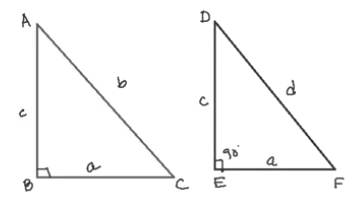In △ABC, AC = b, BC = a, AB = c,

b2 = c2 + a2 — (1)

∠ABC = 90o

In △DEF, DE = AB = c, EF = BC = a, DF = d,

d2 = c2 + a2 — (2)

b2 = d2

b = d

In △ABC and △DEF,

DE = AB = c

EF = BC = a

DF = AC

By SSS congruence,

△ABC ⩭ △DEF

∠DEF = ∠ABC = 90o

So, ∠ABC = 90o

[ii]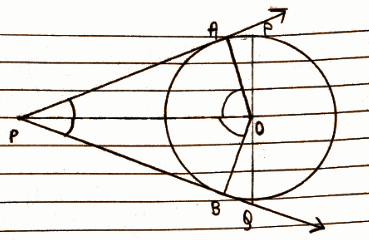In ΔAPO and ΔBPO

AP = BP (theorem)

OP = OP (common)

ΔAPO ≅ ΔBPO (by SSS congruence)

∠AOP = ∠BOP (hence, they subtend equal angle at centre P)

∠APO = ∠BPO (hence, they are equally inclined)

Question 10: Answer any one question: [3 x 1 = 3]

(i) Prove that the quadrilateral formed by the internal bisectors of the four angles of a quadrilateral is cyclic.

(ii) O is the circumcentre of △ABC and OD ⟂ BC; prove that ∠BOD = ∠BAC.

Solution:

[i]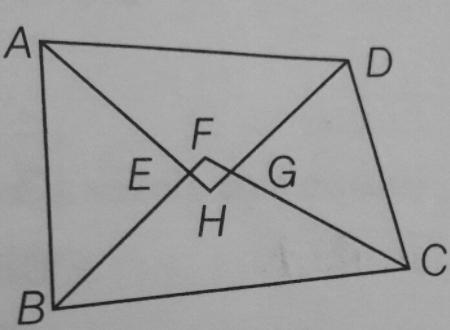Let ABCD be a quadrilateral in which the angle bisectors AH, BF, CF & DH of internal ∠A, ∠B, ∠C & ∠D respectively form a quadrilateral EFGH.

we have to prove that the sum of one pair of opposite angles of a quadrilateral is 180°.

∠E + ∠G = 180° OR

∠F +∠H = 180°

In ∆ AEB,

∠ABE + ∠BAE + ∠AEB = 180°

∠AEB = 180°- ∠ABE – ∠BAE

∠AEB = 180°- (1 / 2 ∠B + 1 / 2 ∠A)

∠AEB = 180° – 1 / 2 (∠B + ∠A) ………….(1)

AH & BF are bisectors of ∠A & ∠B.

Lines AH and BF intersect.

∠ FEH = ∠AEB (vertically opposite angles)

∠ FEH = 180° – 1/2 (∠B + ∠A ) ……….(2)

Similarly, ∠FGH = ∠GCD

∠FGH = 180°- (1 / 2) (∠C + ∠ D)…………… (3)

On adding equations (2) and (3)

∠FEH + ∠FGH = 180° – 1 / 2 (∠A + ∠B) + 180° – (1 / 2) (∠C + ∠D)

∠FEH + ∠FGH = 180° + 180° – (1 / 2) (∠A + ∠B + ∠C + ∠D)

∠FEH + ∠FGH = 360° – (1 / 2) (∠A + ∠B + ∠C + ∠D)

∠FEH + ∠FGH = 360° – (1 / 2) x 360°

[∠A + ∠ B + ∠C + ∠ D = 360°, Sum of angles of Quadrilateral is 360°]

∠FEH +∠ FGH = 360° – 180°

∠FEH + ∠ FGH = 180°

Hence, EFGH is a cyclic quadrilateral in which the sum of one pair of opposite angles is 180°.

∠FEH + ∠FGH = 180°

[ii]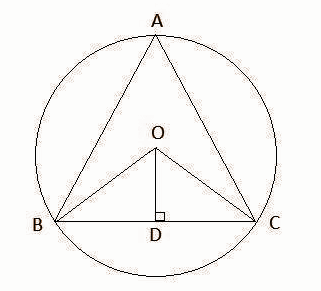O is the circumcenter of ΔABC and OD ⊥ BC

∴ O is the point of intersection of perpendicular bisectors of the sides of ΔABC.

⇒ D is the midpoint of BC

⇒ BD = DC

In ΔOBD and ΔOCD,

OB = OC (Radius of circle)

OD = OD (Common)

BD = DC (Proved)

ΔOBD ≅ ΔOCD (SSS congruence criterion)

⇒ ∠BOD = ∠COD (By CPCT )

∠BOD = ∠COD = (1 / 2) ∠BOC —- (1)

The angle subtended by an arc at the centre is double the angle subtended by it at any point on the remaining part of the circle.

⇒ ∠BOC = 2∠BAC

⇒ 2∠BOD = 2∠BAC (From equation (1))

⇒ ∠BOD = ∠BAC

Question 11: Answer any one question: [5 x 1 = 5]

(i) Draw an equilateral triangle of side 6 cm and draw the incircle of the triangle (only traces of construction are required.)

(ii) Construct a rectangle with sides 8 cm and 6 cm and construct a square equal in area to that of the rectangle (only traces of construction are required.)

Solution:

[i]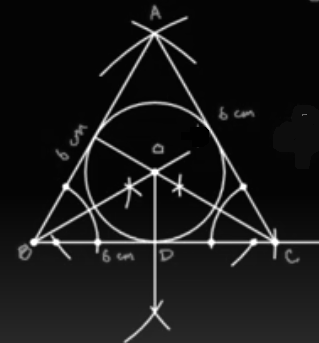[ii]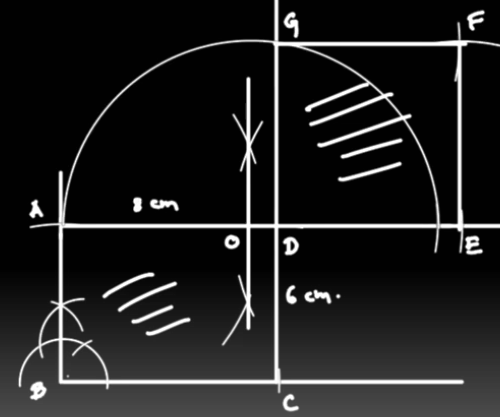Question 12: Answer any two questions: [3 x 2 = 6]

[i] If the measures of three angles of a quadrilateral are 𝛑 / 3, 5𝛑 / 6 and 90o, then determine the fourth angle in sexagesimal and circular measure.

[ii] If sin θ / x = cos θ / y then prove that sin θ – cos θ = [x – y] / √x2 + y2.

[iii] If tan 9o = a / b, that prove that sec2 81o / 1 + cot2 81o = b2 / a2.

Solution:

[i] 𝛑 / 3 = 180 / 3 = 60o

5𝛑 / 6 = 5 * 180 / 6 = 150o

Sum of the angles = 60 + 150 + 90 = 300o

Sum of the angles of a quadrilateral = 360o

Fourth angle = 360o – 300o = 60o

180 = 𝛑

60 = 𝛑 / 180 * 60

= 𝛑 / 3

[ii] sin θ / x = cos θ / y

sin θ / cos θ = x / y

tan θ = x / y

cos θ = ± y / √x2 + y2

sin θ / cos θ = tan θ

sin θ = tan θ * cos θ

LHS = sin θ – cos θ

= tan θ * cos θ – cos θ

= cos θ (tan θ – 1)

= ± y / √x2 + y2 * (x / y – 1)

= [x – y] / √x2 + y2

[iii] tan 9o = a / b

sec2 81o / 1 + cot2 81o

= sec2 81o / cosec2 81o

= (1 / cos2 81o) / (1 / sin2 81o)

= tan2 81o

= cot (90o – 81o)2

= (cot 9o)2

= (1 / tan 9o)2

= 1 / (a / b)2

= b2 / a2

Question 13: Answer any one question: [5 x 1 = 5]

(i) The distance between the two pillars is 150m. Height of one is thrice the other. From the midpoint of the line segment joining the foot of the pillars, the angle of elevation of the top of the pillars is complementary to each other. Find the height of the shorter pillar.

(ii) If the angle of depression from a lighthouse of two ships situated in the same line with the lighthouse is 60° and 30° and if the nearer ship is 150 m away from the lighthouse, then find the distance of the other ship from the lighthouse.

Solution:

[i]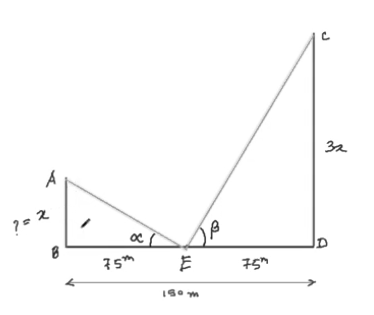ɑ + β = 90o

AB = x m

CD = 3x m

BE = DE = BD / 2 = 150 / 2 = 75 m

In △ABE,

tan ɑ = AB / BE

tan ɑ = x / 75

x = 75 tan ɑ

In △CDE,

tan β = CD / DE

= 3x / 75

= 3 * (x / 75)

= 3 * tan ɑ

tan (90o – ɑ) = 3 tan ɑ

cot ɑ = 3 tan ɑ

1 / tan ɑ = 3 tan ɑ

3 tan2 ɑ = 1

tan2 ɑ = 1 / 3

tan ɑ = 1 / √3

x = 75 tan ɑ

x = 75 * (1 / √3)

x = 25√3 m

[ii]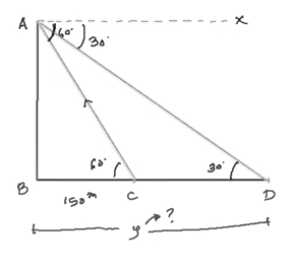AB = x m

BC = 150m

BD = y m

AX || BD

∠XAC = ∠BCA = 60o

In △ABC,

tan 60o = x / 150

√3 = x / 150

x = 150√3m

In △ABD,

tan 30o = AB / BD

1 / √3 = x / y

y = √3x

y = √3 * (150√3)

y = 450m

Question 14: Answer any two questions: [4 x 2 = 8]

(i) Determine the volume of a solid right circular cone which can be made from a solid wooden cube of 4.2 dcm edge length by wasting a minimum quantity of wood.

(ii) A hemispherical bowl with a radius of 9 cm is completely filled with water. How many cylindrical bottles of diameter 3 cm and height 4 cm can be filled, up with the water in the bowl?

(iii) Area of the base of a closed cylindrical water tank is 616 square meter and the height is 21 meter. Find the total surface area of the tank.

Solution:

[i] The volume of the cone should be maximum

∴ The radius of the base of the cone: = edge of cube / 2

= 4.2 / 2

= 2.1 dm

Height of cone = edge of cube = 4.2 dm.

∴ The volume of the cone = [1 / 3] πr2h

= (1 / 3 × 22 / 7 × 2.1 × 2.1 × 4.2) cu.dm.

= 58.1 cu.dm

[ii] Volume of hemispherical bowl = Volume of one cylindrical shaped ball

R = 9cm

r = 3 / 2 cm

h = 4cm

(2 / 3) 𝛑R3 = x * (𝛑r2h)

(2 / 3) * 93 = x * (3 / 2)2 * 4

486 = x * 9

x = 486 / 9

x = 54 bottles

[iii] Area of base = 616 sq. m

Let the radius be x metre

Area of circle = 616 sq. m

πr2 = 616

22 / 7 * r * r = 616

r * r = 616 * 7 / 22

= 28 * 7

=196

r = √196

r = 14 m

Height of cylinder = 21 m

Radius of cylinder = 14 m

Total surface area of cylinder = 2πr (r + h)

= 2 * 22 / 7 * 14 (14 + 21)

= 2 * 22 * 2 * 35

= 88 * 35

= 3080 sq. m

Question 15: Answer any two questions: [2 x 4 = 8]

[i] If the median of the following data is 32, find the values of x and y when the sum of the frequencies is 100.

 Class Interval Frequency 0 – 10 10 10 – 20 x 20 – 30 25 30 – 40 30 40 – 50 y 50 – 60 10

[ii] Find the mode from the following distribution table:

 Class Interval Frequency 0 – 5 5 5 – 10 12 10 – 15 18 15 – 20 28 20 – 25 17 25 – 30 12 30 – 35 8

[iii] By preparing a cumulative frequency (greater than type) table from the following data, draw an ogive in a graph paper.

 Class Interval Frequency 0 – 5 4 5 – 10 10 10 – 15 15 15 – 20 8 20 – 25 3 25 – 30 5

Solution:

[i]
 Class Interval Frequency CF 0 – 10 10 10 10 – 20 x 10 + x 20 – 30 25 35 + x 30 – 40 30 65 + x 40 – 50 y 65 + x + y 50 – 60 10 75 + x + y

h / 2 = 50

75 + x + y = 100

x + y = 25 —- (1)

f = 30

c = 10

CF = 35 + x

L = 30

Median = L + [(N / 2 – CF) / f ]* c

32 = 30 + [(50 – 35 – x) / 30] * 10

32 – 30 = 50 – 35 – x / 3

6 – 15 = -x

x = 9

Substitute the value of x in (1),

9 + y = 25

y = 16

[ii] Mode = Z = L1 + (F1 – F0) / (2F1 – F0 – F2) * i

= 15 + [28 – 18] / [2 * 28 – 18 – 17] * 5

= 15 + 10 / 21 * 5

= 15 + 2.38

= 17.38

[iii]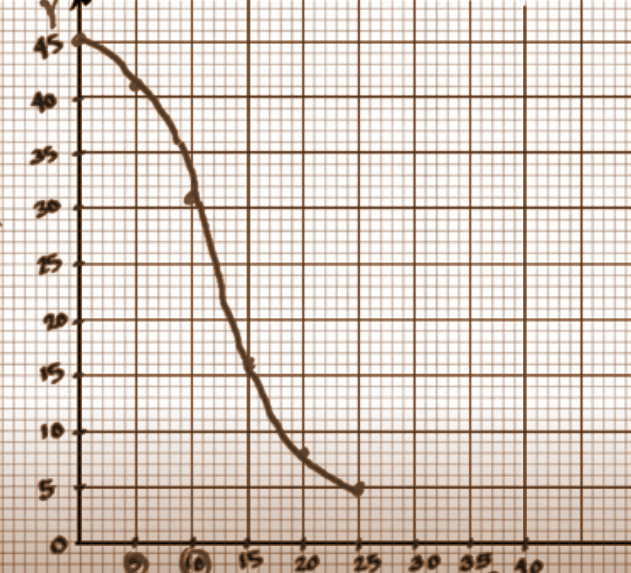[Alternative Question for Sightless Candidates]

Question 11: Answer any one question: [5 x 1 = 5]

(i) Describe the procedure of drawing the incircle of an equilateral triangle whose side is given.

(ii) Describe the procedure of construction of a square of the equal area of a rectangle whose sides are given.

Solution:

An example of drawing the incircle of an equilateral triangle whose side is 8 cm is explained below.

[i] An equilateral triangle ABC in which AB = BC = CA = 8cm.

Construction of △ABC

(1) Draw a line segment BC = 8cm

(2) Draw an arc of radius = 8cm taking B as the centre.

(3) Draw another arc of radius = 8cm taking B as centre

(4) Draw another arc of radius = 8cm taking C as the centre with intersects the previous arc at a point A.

(5) Join AB and AC.

Thus the required ABC is constructed.

Construction of incircle of △ABC

(1) Draw BP and CQ the bisectors of angles, ∠B and ∠C respectively and which intersect each other at point C.

(2) Draw OR perpendicular to BC which intersects BC at L.

(3) Taking O as the centre and OL as radius draw a circle which touches the sides AB, BC and CA at points N, L and M, respectively.

(4) Thus the required incircle is constructed.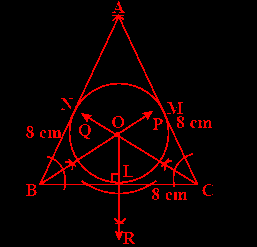[ii]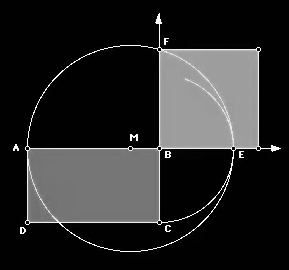• Extend side AB.
• Draw an arc with B as the centre and with radius BC. Let E be the intersection of the arc and ray AB.
• Construct the midpoint M of segment AE.
• Construct a circle with centre at M with radius MA.
• Extend side CB.
• Let F be the point where the circle and ray CB intersect.
• Then BF is the side of the desired square.
• Complete by constructing a square with side BF.

Question 16: (a) Answer any three questions: [3 x 2 = 6]

(i) If x ∝ y, y ∝ z and z ∝ x then find the relation between the constants of variations.

(ii) If in the case of compound interest, the rate of interest in 1st, 2nd and 3rd year are r1%, r2% and r3%, respectively, then find the amount after 3 years for Rs P.

(iii) If √2 sin (2x + 5°) = tan 45°, find the value of sec 3x.

(iv) What is the ratio of the surface area of a solid sphere and a solid hemisphere of equal radius?

Solution:

[i] x ∝ y, y ∝ z and z ∝ x

It can be written as,

x = ky, y = mz and z = nx where k, m and n are constants of variations.

Put the value of y in x,

x = kmz

Now put the value of z in x,

⇒ x = kmnx

⇒ kmn = 1

[ii] Amount = P * [1 + (r1 / 100)] * [1 + (r2 / 100)] * [1 + (r3 / 100)] [iii] √2 sin (2x + 5°) = tan 45°

√2 sin (2x + 5°) = 1

sin (2x + 5°) = 1 / √2

(2x + 5o) = sin-1 (1 / √2)

2x + 5 = 45

2x = 40

x = 40 / 2

x = 20

sec (3 * 20) = sec 60o = 2

[iv] The radius of both the hemisphere and sphere be ‘r’.

The surface area of the sphere = [4 / 3]𝛑r3

The surface area of the hemisphere = [2 / 3]𝛑r3

The ratio of the total surface area of a sphere and a solid hemisphere of the same radius

= [4 / 3]𝛑r3 / [2 / 3]𝛑r3

= 2:1

(i) If (a + 2b) : (3a – 2b) = 9 : 13, then find the value of a : b.

(ii) What should be subtracted from √72 to get √32?

(iii) What is the length of the tangent drawn from a point at a distance of 13 cm from the centre of a circle whose radius is 5 cm?

(iv) Find the mean proportion of 3 and 12.

(v) Justify that the roots of the equation 4x2 – 4x + 1 = 0 are real and equal.

Solution:

[i] (a + 2b) : (3a – 2b) = 9 : 13

a + 2b / 3a – 2b = 9 / 13

13 * (a + 2b) = 9 * (3a – 2b)

13a + 26b = 27a – 18b

44b = 14a

a = 44 / 14 * b

44 = 14 (a / b)

44 / 14 = a / b

44:14 = a:B

[ii] Let the number be x

√72 – x = √32

6√2 – x = 4√2

x = 6√2 – 4√2

= √2 [6 – 4]

= 2√2

[iii] A tangent is drawn from P which touches the circle at T.

Length of the tangent is PT and the centre of the circle is O.

PO = 13cm , OT = radius = 5cm

The tangent is perpendicular to the radius of the circle.

∆POT is a right-angled triangle.

PT² + OT² = OP²

PT² + 5² = 13²

PT² = 169 – 25 = 144

PT = 12cm

The length of tangent = 12cm.

[iv] Let the mean proportion between 12 and 3 be a.

= > 12 / a = a / 3

= > 12 x 3 = a x a

= > 4 x 3 x 3= a2

= > 6 x 6 = a2

= > 6 = a

Therefore, the mean proportion between 12 and 3 is 6.

[v] 4x2 – 4x + 1 = 0

On comparing the given equation to ax2 + bx + c = 0,

a = 4, b = – 4, c = 1

The condition for the roots to be real and equal is b2 – 4ac = 0.

(-4)2 – 4 * 4 * 1 = 16 – 16 = 0

So, the roots are real and equal.